﻿ 重新找回人工智能的可解释性
«上一篇文章快速检索 高级检索

 智能系统学报2019, Vol. 14Issue (3): 393-412  DOI: 10.11992/tis.2018100200

### 引用本文HE Huacan. Refining the interpretability of artificial intelligence[J]. CAAI Transactions on Intelligent Systems, 2019, 14(3): 393-412. DOI: 10.11992/tis.201810020.### 文章历史

Refining the interpretability of artificial intelligence
HE HuacanSchool of Computer Science, Northwestern Polytechnical University, Xi’an 710072, China
Abstract: In view of the restrictions on the interpretability of artificial intelligence (AI) research on deep neural networks, it is indicated that rigid logic (mathematical formal logic) and binary neurons are equivalent. Moreover, a binary neural network can be converted into a logical expression, which is highly interpretable. The deep neural network blindly increases the number of intermediate layers to fit big data without the timely abstraction of data with the smallest granularity (atom) into knowledge with larger granularity (molecule), changes knowledge with smaller granularity into knowledge with larger granularity, and submerges the original strong explanatory power in the ocean of intermediate layers. To support knowledge processing of multiple granularities, rigid logic should be expanded into flexible propositional logic (proposition-level mathematical dialectic logic) and binary neurons should be expanded into flexible neurons to maintain the strong explanatory power. This paper introduces in detail the achievement of the expansion process from rigid logic to flexible logic and its application in AI research, which is the best method to recover the interpretability of AI.
Key words: artificial intelligence    interpretability    evolution    uncertainty    universal logic    flexible propositional logic    flexible neurons    mathematical dialectic logic

1 现今的人工智能研究何以会失去可解释性 1.1 原本二值神经元与布尔逻辑算子是完全等价的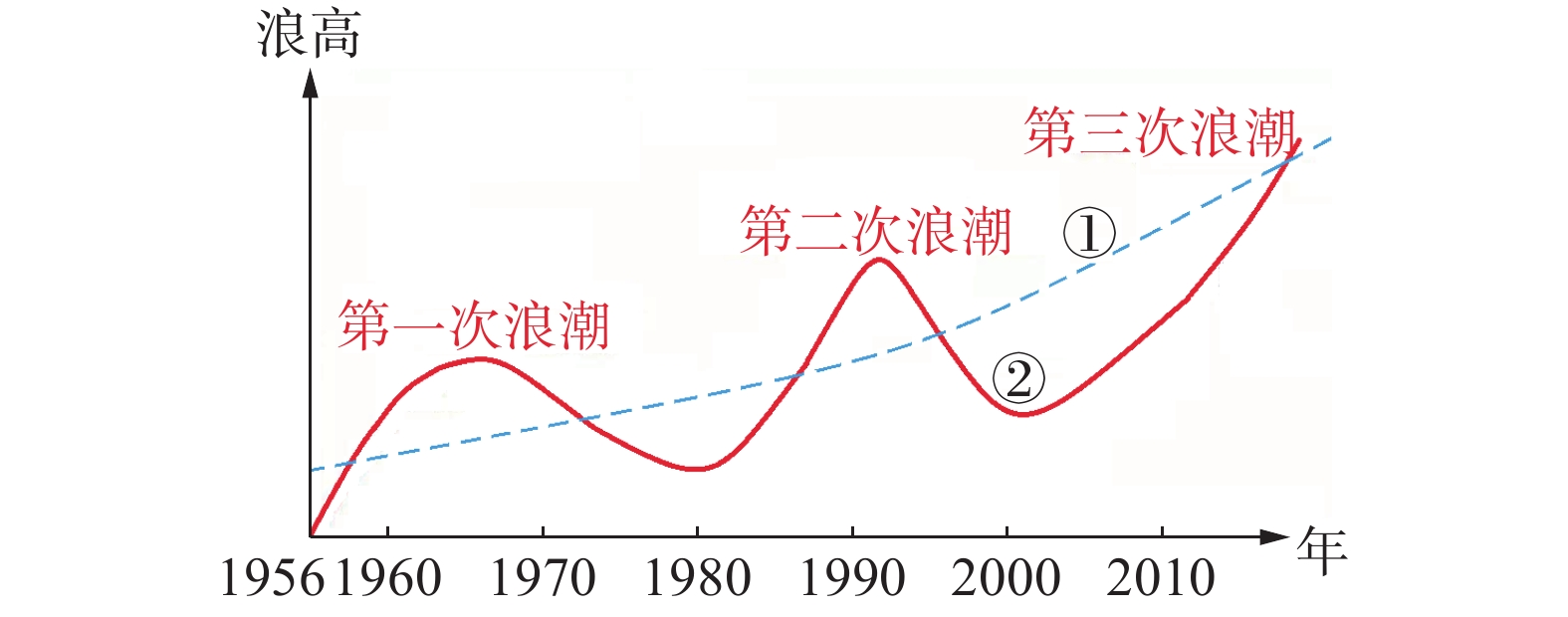Download: 图 1 人工智能学科和产业60年发展态势图 Fig. 1 Development trend of the AI discipline and industry in 60 years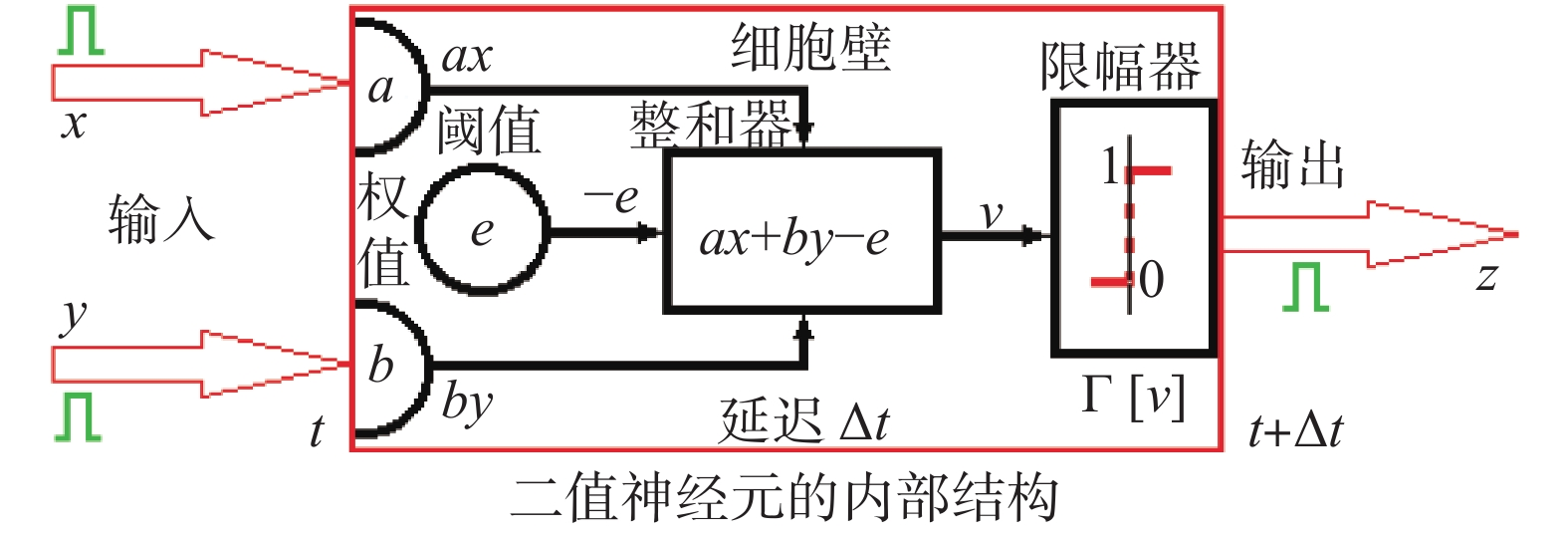Download: 图 2 二值神经元的MP模型 Fig. 2 MP model of two valued neurons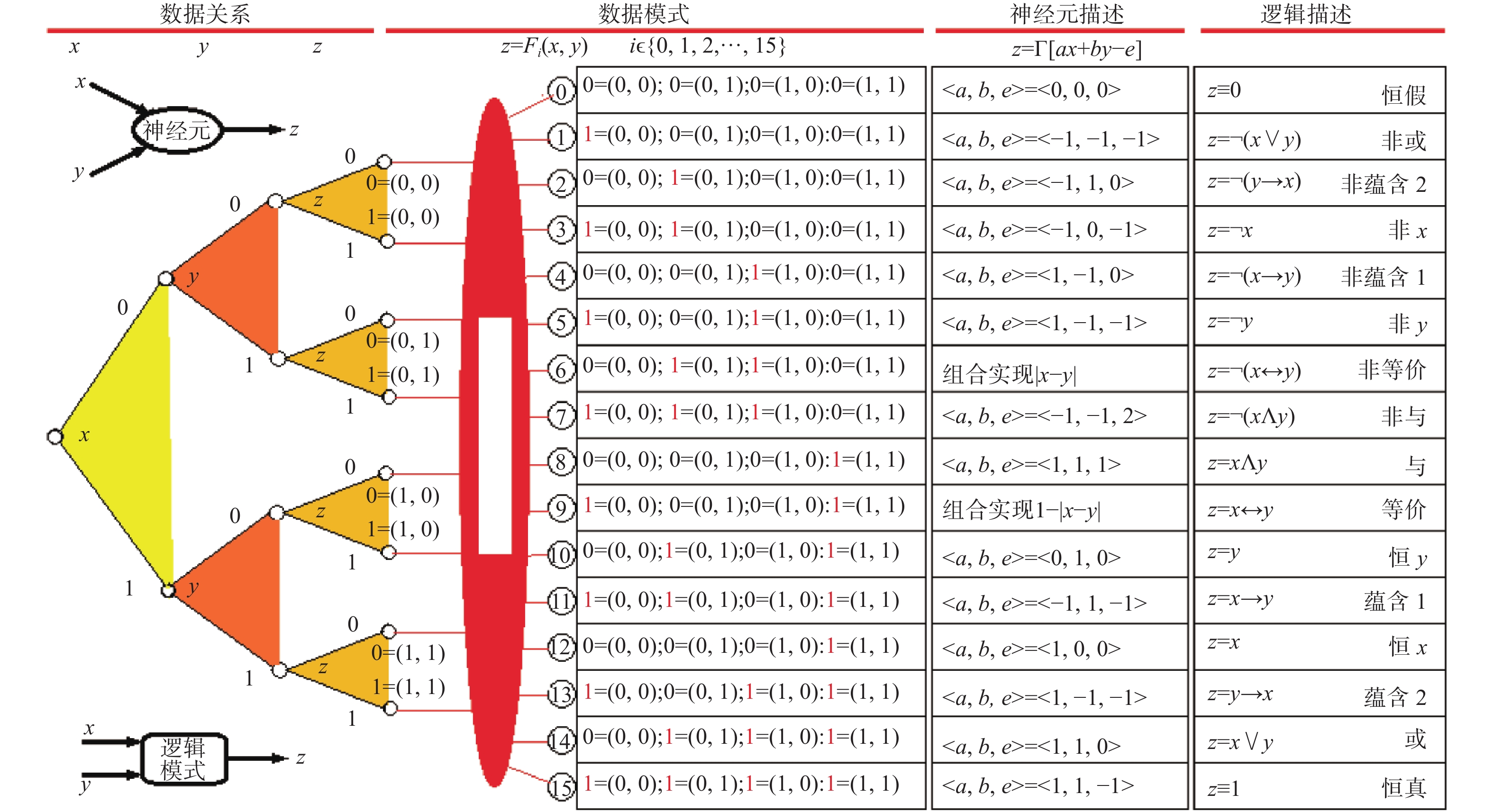Download: 图 3 刚性逻辑算子和MP神经元模型等价 Fig. 3 Equivalence of the rigid logic operator and MP neuron model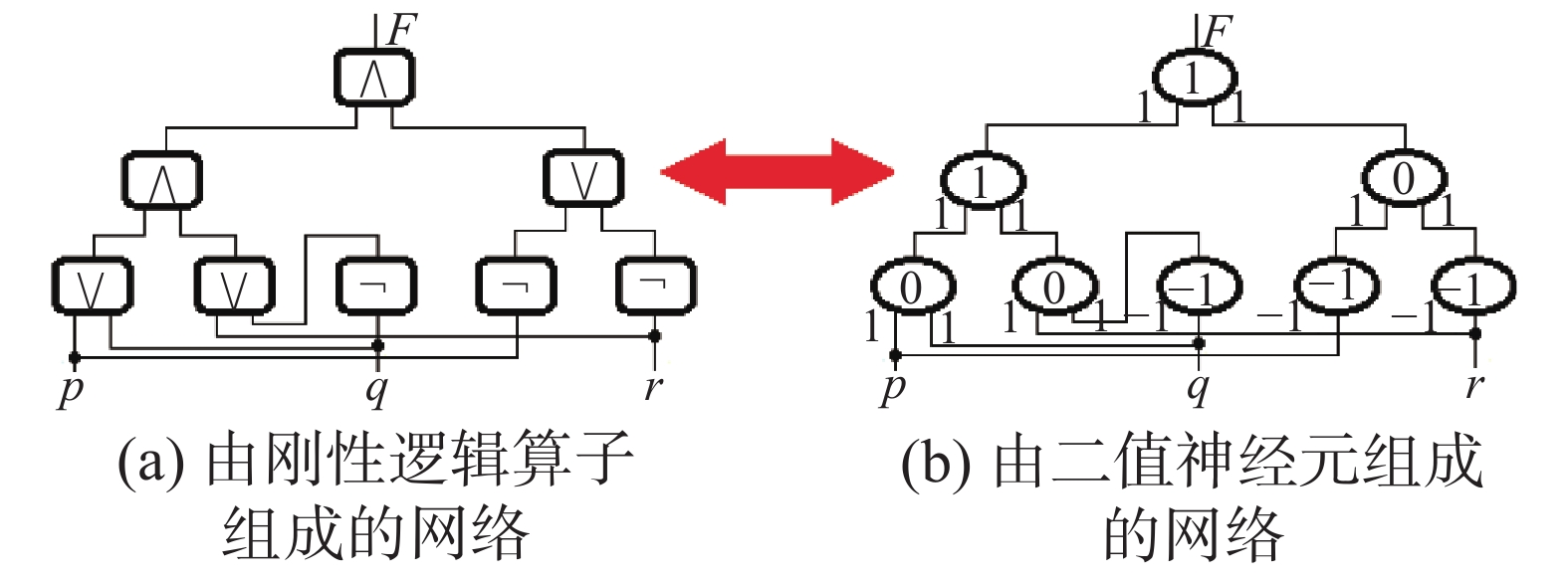Download: 图 4 逻辑网络和神经网络等价的一个实例 Fig. 4 An example of the equivalence between logical and neural networks
1.2 当前人工智能研究失去可解释性的原因

20世纪80年中期代爆发的人工智能“理论危机”无情地揭露了刚性逻辑、启发式搜索原理和经验知识推理的应用局限性：首先，刚性逻辑本身的推理效率十分低下，如果没有启发式知识的引导，单纯机械式地按照刚性逻辑的规则进行推理，算法的指数复杂度必然带来组合爆炸，计算机的时空资源迅速被吞噬殆尽；其次，在启发式搜索和经验知识推理中，客观存在的各种不确定性和演化过程都超出了刚性逻辑的有效适用范围，尽管出现了一些非标准逻辑(如模糊逻辑、概率逻辑和有界逻辑等)能解决某些实际问题，但有时会出现违反常识的异常结果，这说明非标准逻辑在理论上并不成熟可靠，无法在人工智能中安全可靠地使用。要有效解决包含各种不确定性和演化的现实问题，只能寄希望于尽快建立数理辩证逻辑理论体系，可是在当时的情况下，学术界的思想和理论准备都严重不足，建立数理辩证逻辑谈何容易！

“物极必反”是自然的一条重要发展规律，在盲目乐观思潮弥漫的今天，已有一些著名的人工智能学者在讨论现有人工智能面临的局限性：1)有智能没有智慧，无意识和悟性，缺乏综合决策能力；2)有智商没有情商，机器对人的情感理解与交流还处于起步阶段；3)会计算不会“算计”，人工智能可谓有智无心，更无人类的谋略；4)有专才无通才，会下围棋的不会下象棋。归纳起来说，目前人工智能发展正面临着六大发展瓶颈：1)数据瓶颈，需要海量的有效数据支撑；2)泛化瓶颈，深度学习的结果难于推广到一般情况；3)能耗瓶颈，大数据处理和云计算的能耗巨大；4)语义鸿沟瓶颈，在自然语言处理中存在语义理解鸿沟；5)可解释性瓶颈，人类无法知道深度神经网络结果中的因果关系；6)可靠性瓶颈，无法确认人工智能结果的可靠性。由此可知，人工智能的发展正面临又一次的发展瓶颈，本文统称为“可解释性瓶颈”。这些应用局限性和发展瓶颈对于人类智能来说并不明显存在，为什么却在当今的人工智能研究中成了难以逾越的巨大困难？笔者认为这些困难是由无视逻辑和知识在智能中的重要价值，过度依赖数据统计和深度神经网络引起的。

2011年图灵奖得主Judea Pearl是曾在20世纪80年代推动机器以概率(贝叶斯网络)方式进行推理的领头人，现在他却指出：深度学习所取得的所有成就都只是根据(有效)数据进行的曲线拟合，AI已陷入概率关联泥潭，它不能完全体现智能的真正含义。跳出泥潭的关键措施是用因果推理来代替关联推理，在AI中一旦因果关系就位，机器就有可能提出反事实问题，询问因果关系在某些干预下会如何变化，这才是科学思考的基础。所以只有因果推理才能使机器具有类人智能，有效地与人类交流互动。也只有这样，机器才能获得道德实体的地位，具有自由意志和运用人类谋略的能力。

1.3 重温人类智慧的两个重要特征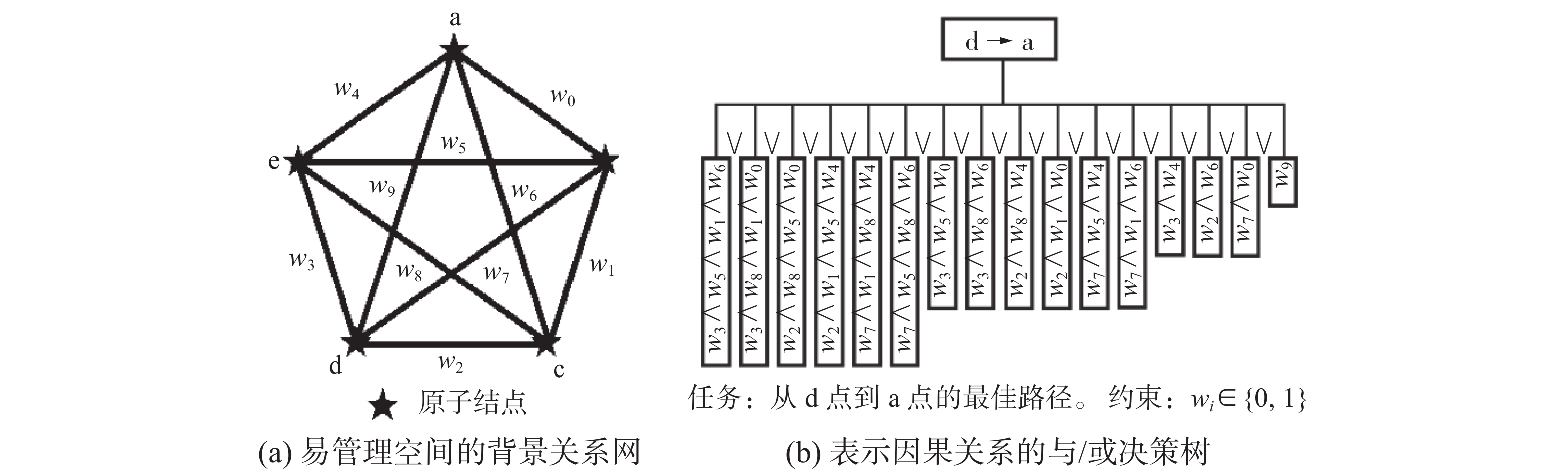Download: 图 5 村落地图和与/或决策树 Fig. 5 Village map and AND/OR decision tree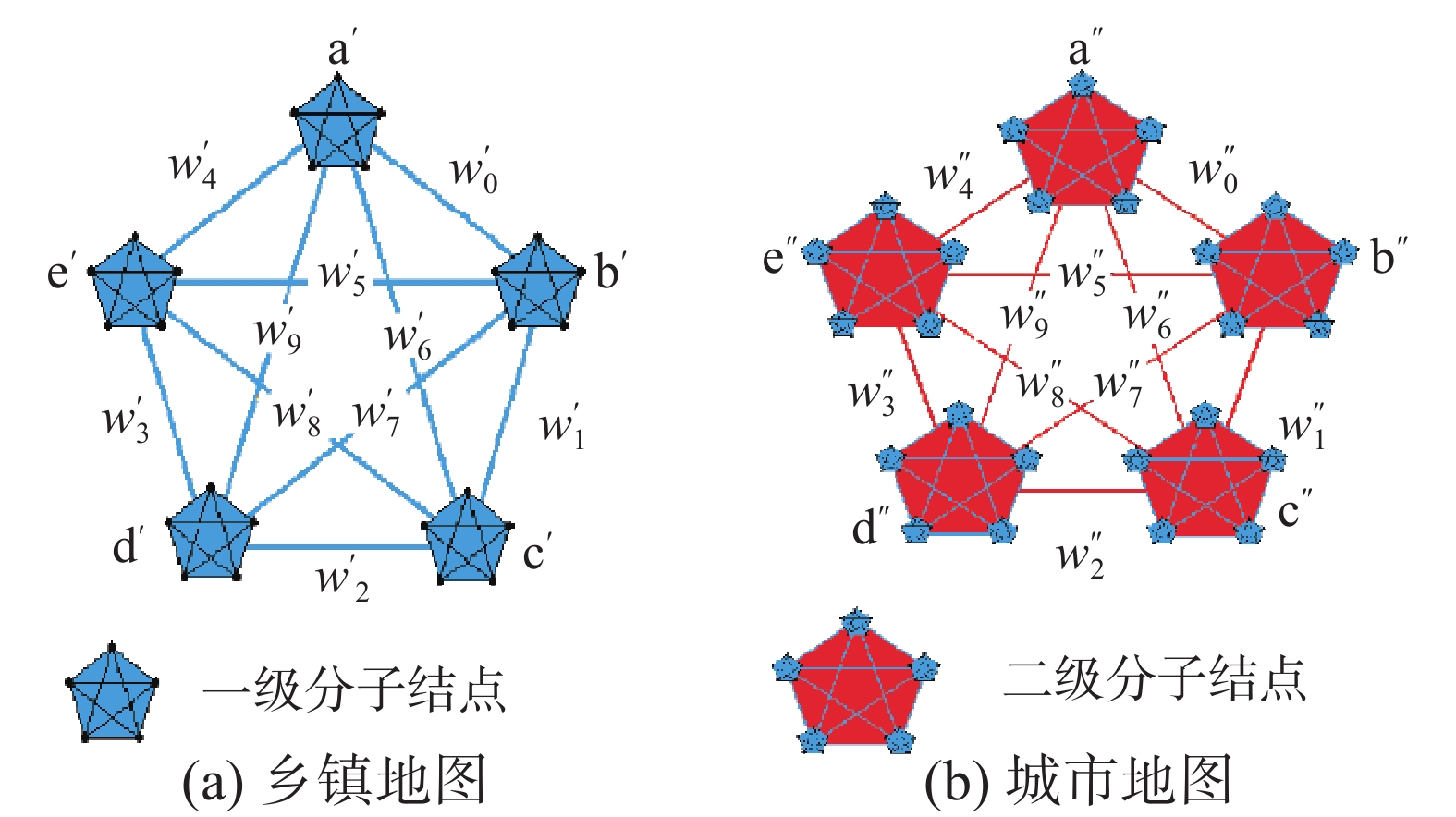Download: 图 6 乡镇地图和地市地图的简化表示 Fig. 6 Simplified representation of district map and municipal map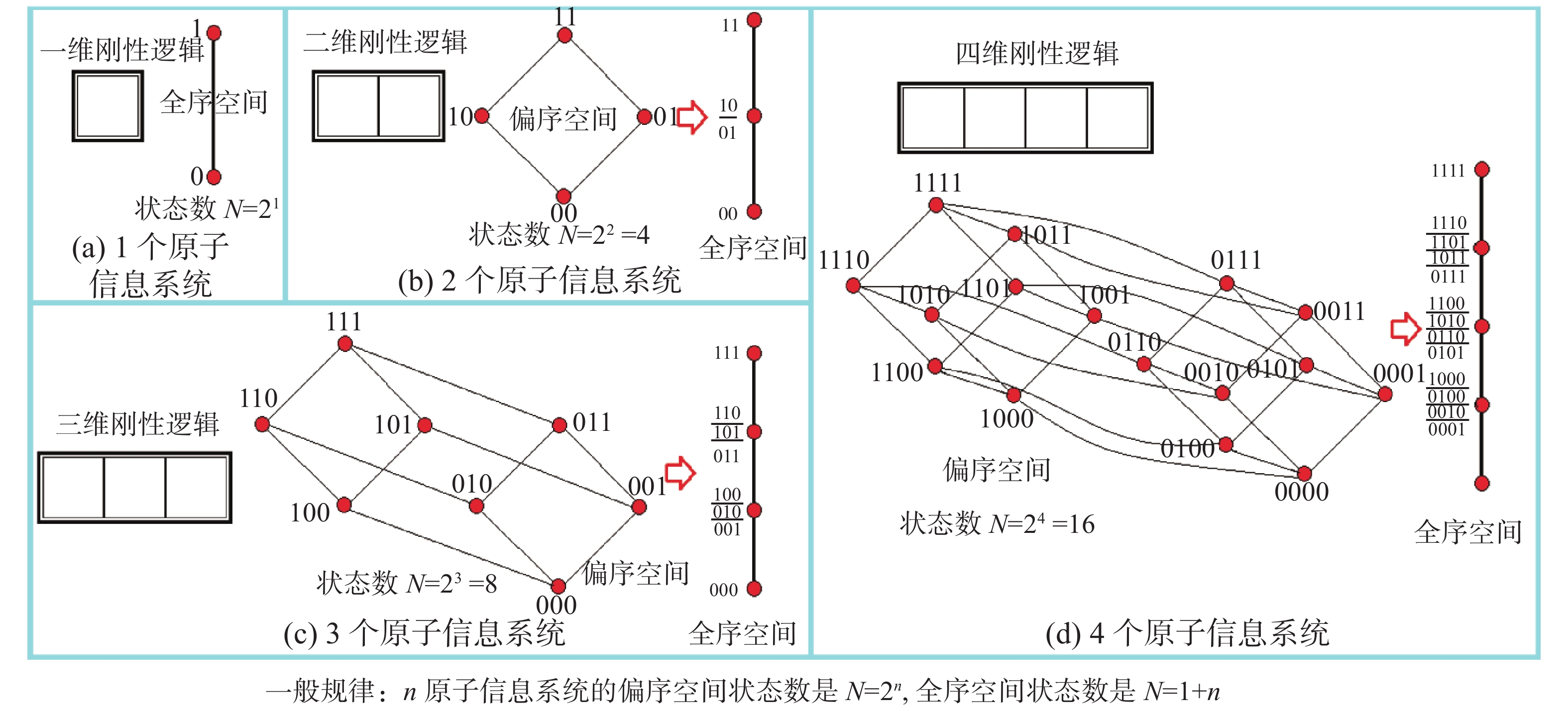Download: 图 7 从确定的原子状态进入不确定性的分子状态 Fig. 7 From the determined atomic state to the molecular state of uncertainty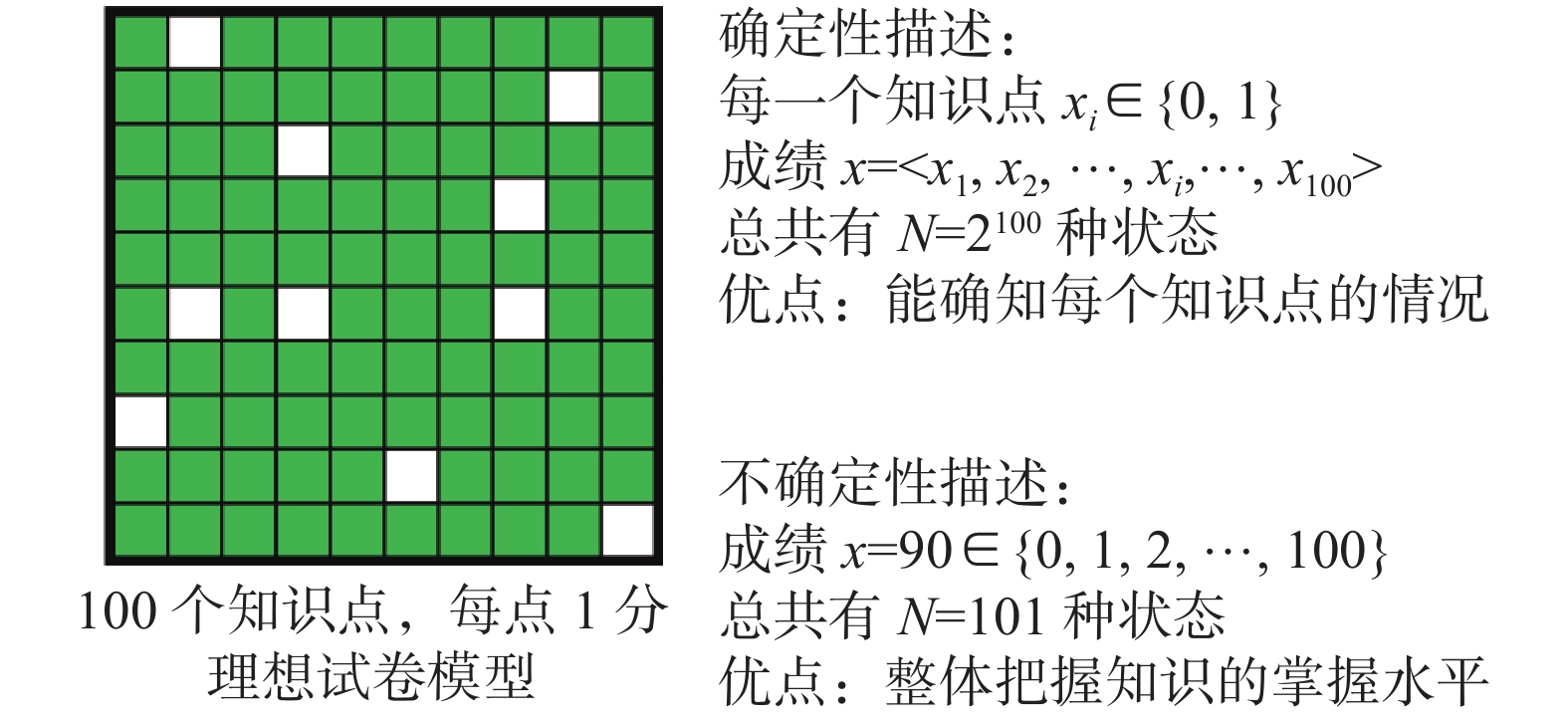Download: 图 8 从试卷模型看确定性和不确定性的关系 Fig. 8 A test paper model is used to illustrate the relationship between certainty and uncertainty

2 从刚性信息处理到柔性信息处理的扩张过程和已有结果 2.1 柔性命题真度的确定方法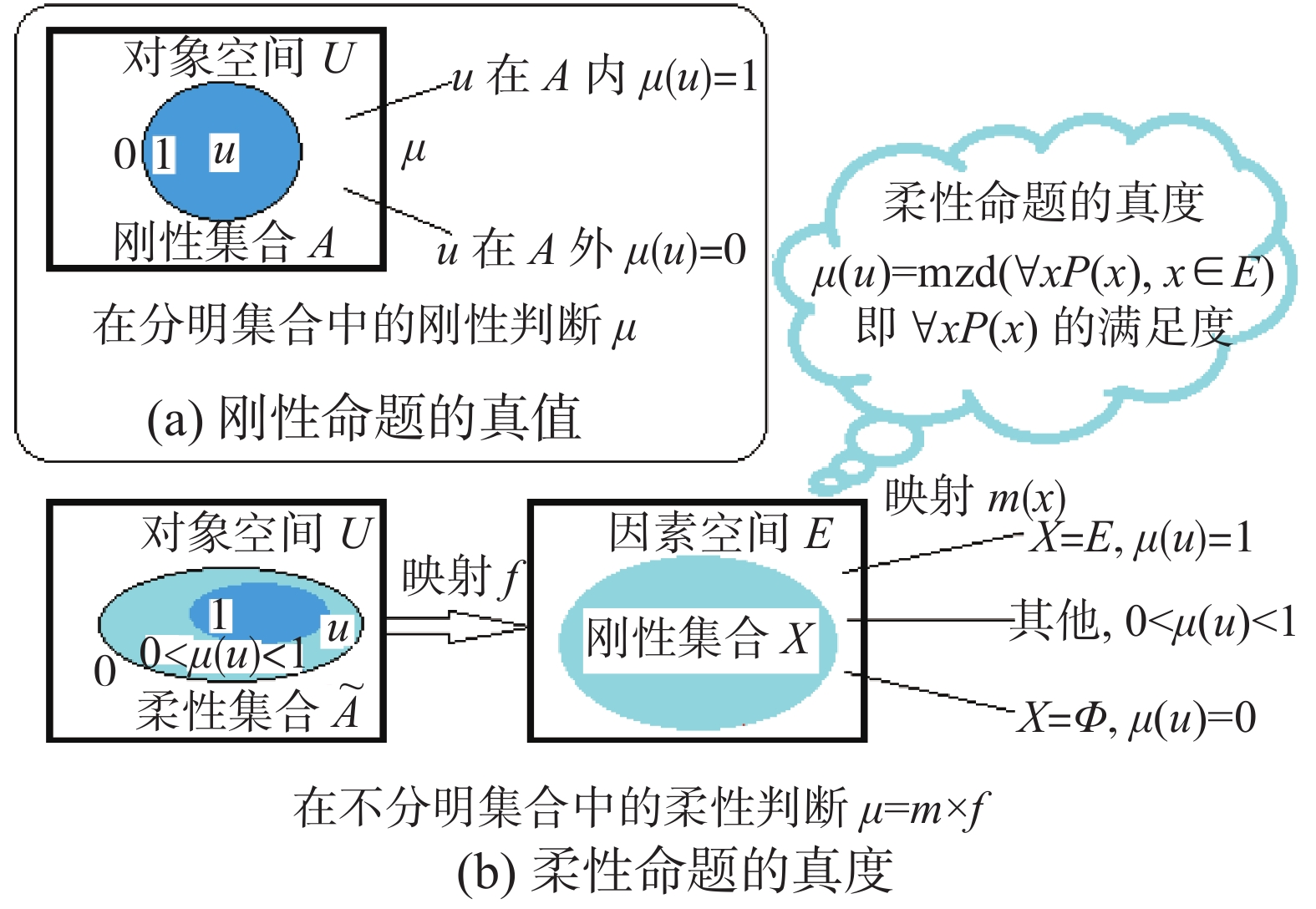Download: 图 9 柔性命题真度的逻辑意义 Fig. 9 Logical meaning of the truth degree of the flexible proposition

 $\mu (u) = {\rm{mzd}}(\forall xP(x),\;x \in E)$

E中谓词公式 $\forall$ xP(x)的满足度。

2.2 完成柔性信息处理扩张的总路线图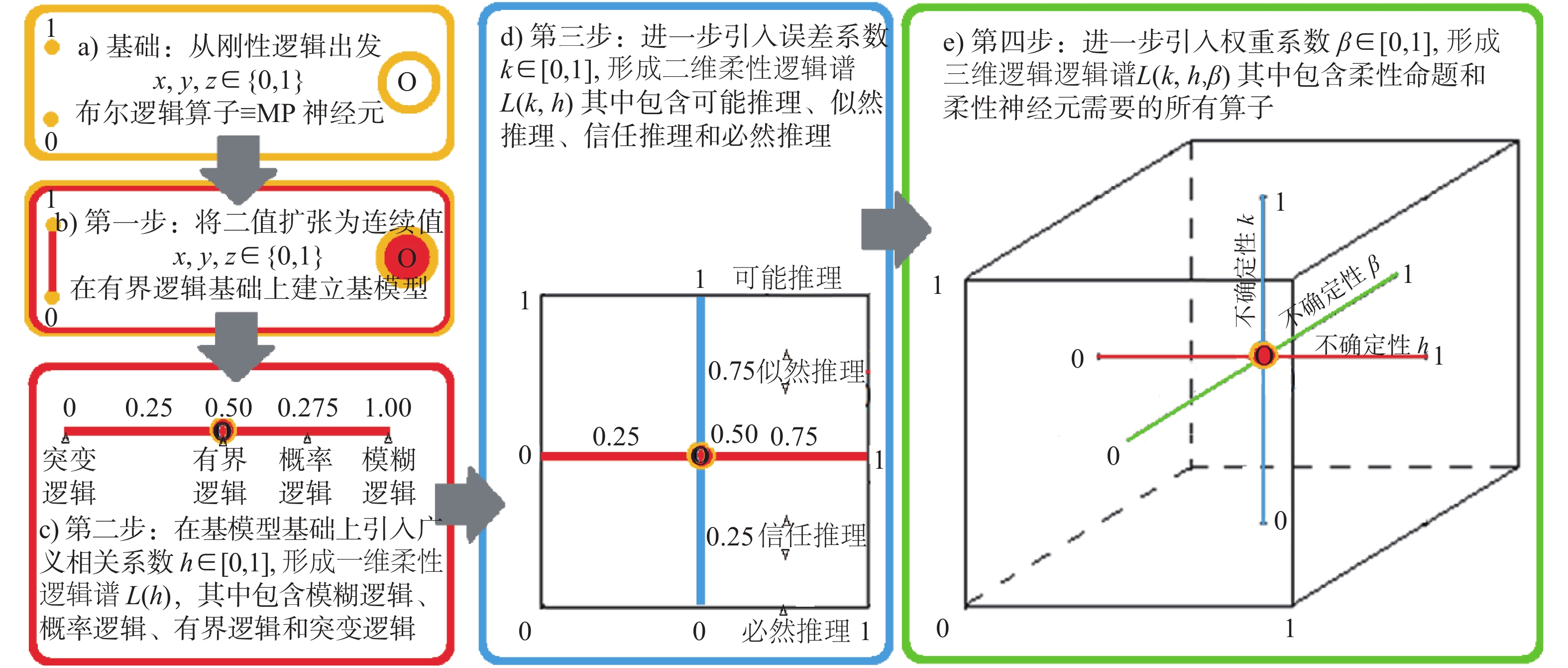Download: 图 10 从刚性逻辑到柔性逻辑的扩张总路线图 Fig. 10 General line map from rigid logic to flexible logic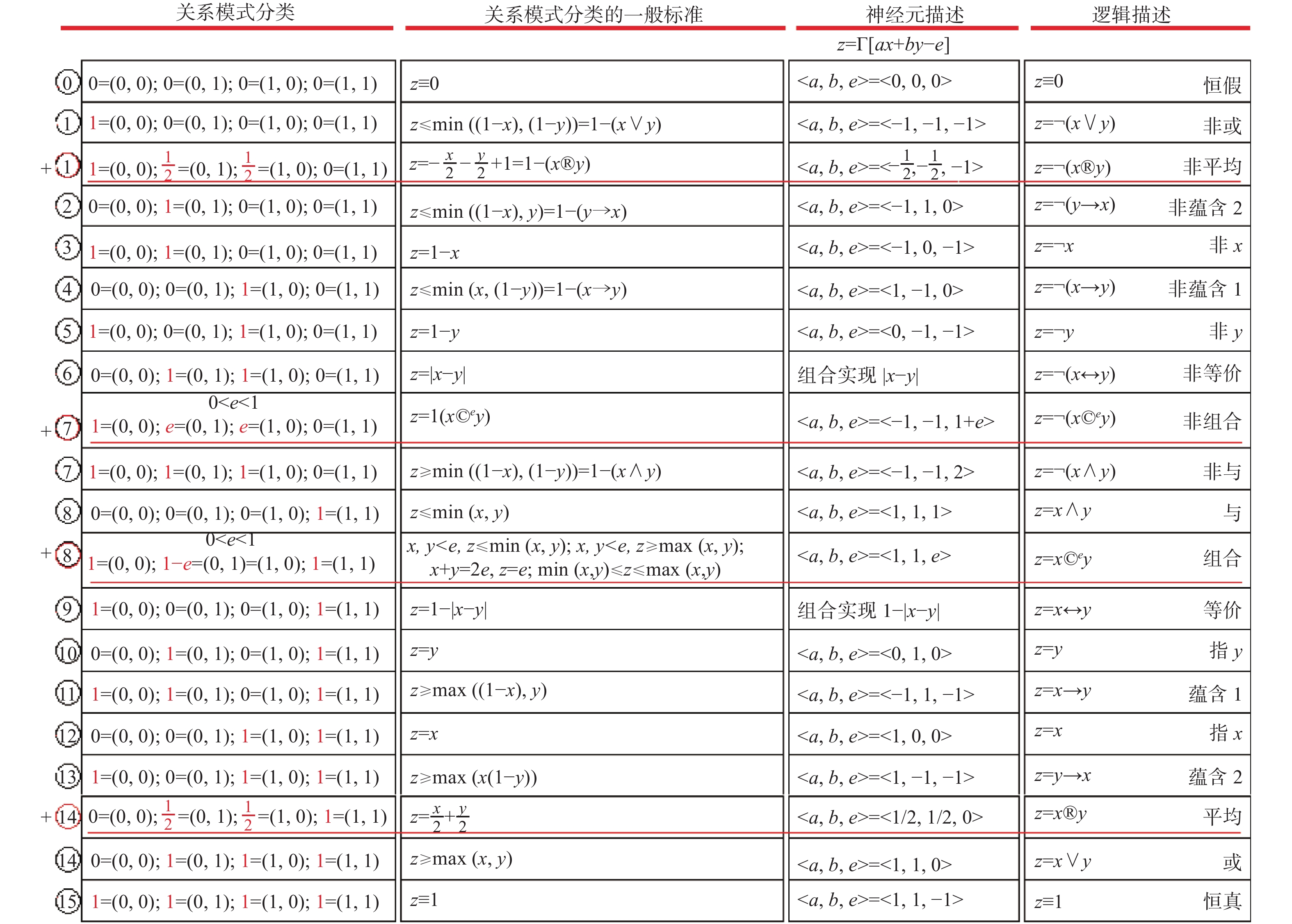Download: 图 11 柔性信息处理中的20种基本模式 Fig. 11 The 20 basic modes in flexible information processing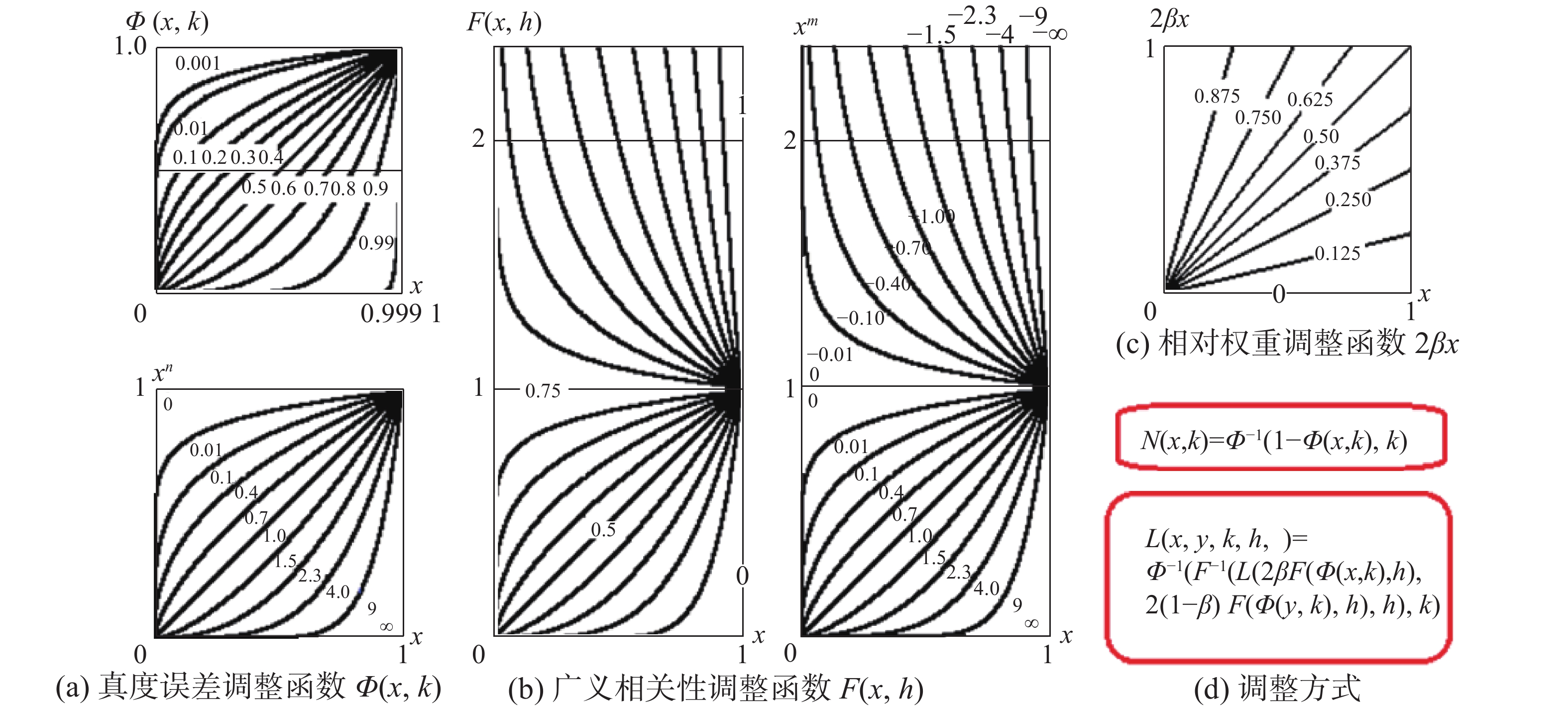Download: 图 12 3种不确定性参数及其调整函数 Fig. 12 Three kinds of uncertainty parameters and their adjustment functions

1)命题真度的误差系数k∈[0, 1]，其中k=1表示最大正误差，k=0.5表示无误差，k=0表示最大负误差。k对基模型的影响完全反映在N性生成元完整簇Φ(x, k)=xnn∈(0, ∞)上，其中n=−1/log2k。当n→0时，Φ(x, 0)=ite{0|x=0; 1}; 当n=1时，Φ(x, 0.5)=x; 当n→∞时，Φ(x, 1)=ite{1|x=1; 0}。Φ(x, k)对一元运算基模型N(x)的作用方式是N(x, k)=Φ−1(N(Φ(x, k)), k)，对二元运算基模型L(x, y)的作用方式是L(x, y, k)=Φ−1(L(Φ(x, k), Φ(y, k)), k)。

2)广义相关系数h∈[0, 1]，其中：h=1是最大的相吸关系或者最大的相容关系；h=0.75是独立相关关系；h=0.5是最大的相斥关系或者最小的相容关系，也就是最弱的敌我关系或者最小相克关系；h=0.25是敌我僵持关系；h=0是最强的敌我关系或者最大的相克关系。广义相关系数h对基模型的影响全部反映在T性生成元完整簇F(x, h)=xm, m∈(−∞, ∞)上，其中：m=(3−4h)/(4h(1−h))。当m→−∞时，F(x, 1)=ite{1|x=1; ±∞}; 当m→0时，F(x, 0.75)=1+lgx; 当m→0+时，F(x, 0.75+)=ite{0|x=0; 1}; 当m=1时，F(x, 0.5)=x; 当m→∞时，F(x, 0)=ite{1|x=1; 0}。

F(x, h)对6种二元运算基模型L(x, y)的影响是

 $L(x,y,h) = F{^{ - 1}}(L(F(x,h),F(y,h)),h)$

3)权系数β∈[0, 1]，其中：β＝1表示最大偏xβ＝0.5表示等权；β＝0表示最小偏x。权系数β对基模型的影响完全反映在二元运算模型上，其对基模型L(x, y)的作用方式是：

 $L(x,y,\beta )=L(2\beta x,2(1-\beta )y)$

k, h, β三者对二元运算模型L(x, y)共同的影响方式是：

 $\begin{split} L(x,y,k,h,\beta ) = & \varPhi{^{ - 1}}(F{^{ - 1}}(L(2\beta F(\varPhi (x,k),h),2(1 - \beta )\cdot \\ & F(\varPhi (y,k),h),h),k) \end{split}$

2.3 柔性信息处理扩张的过程和结果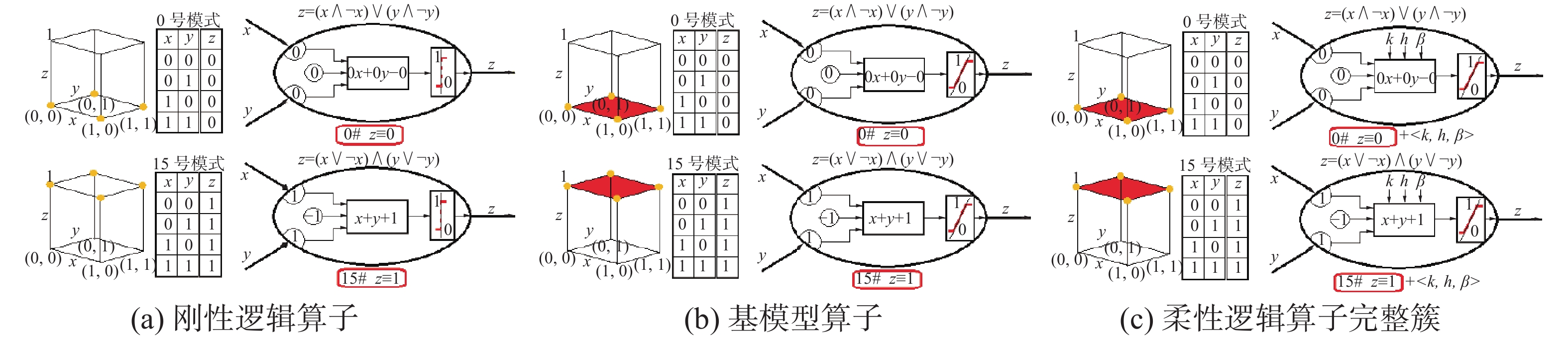Download: 图 13 恒0模式和恒1模式 Fig. 13 Constant 0 pattern and constant 1 pattern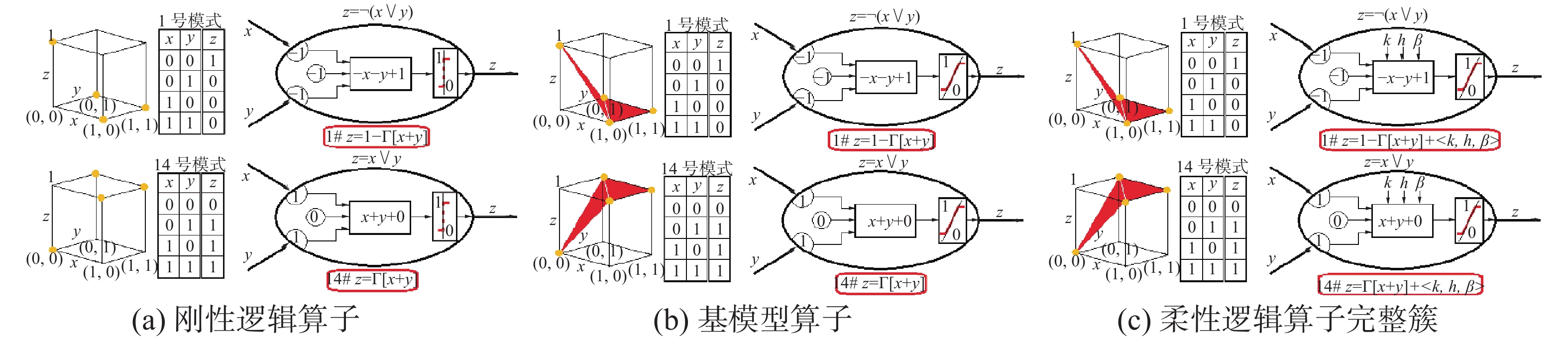Download: 图 14 非或模式和或模式 Fig. 14 N-OR pattern and OR pattern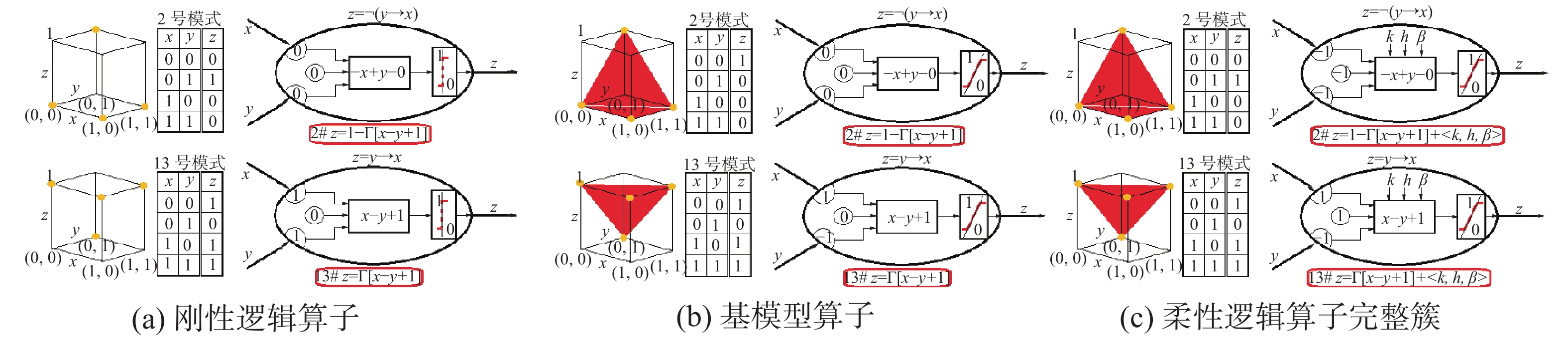Download: 图 15 非蕴涵2模式和蕴涵2模式 Fig. 15 N-IMP-2 pattern and IMP-2 pattern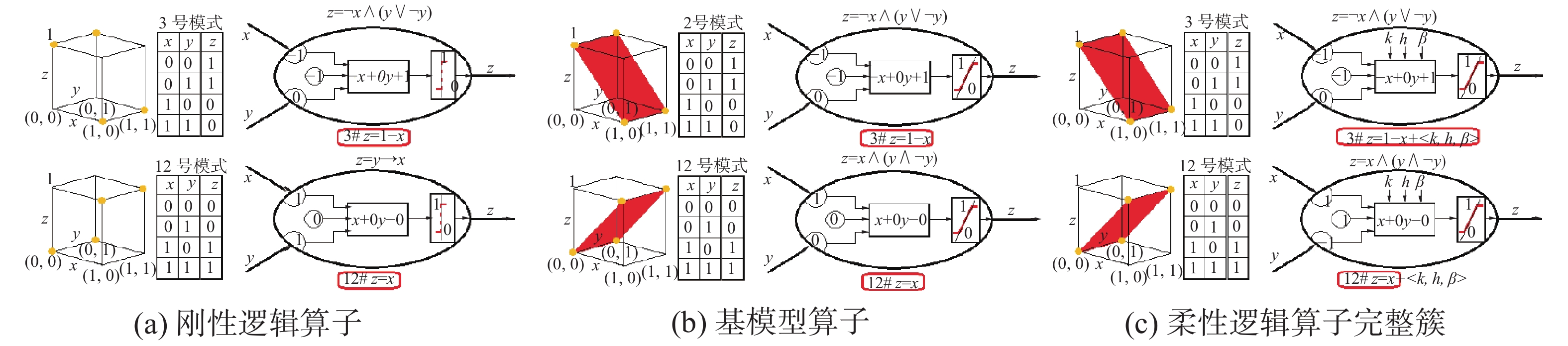Download: 图 16 非x模式和指x模式 Fig. 16 NOT -x pattern and IND -x pattern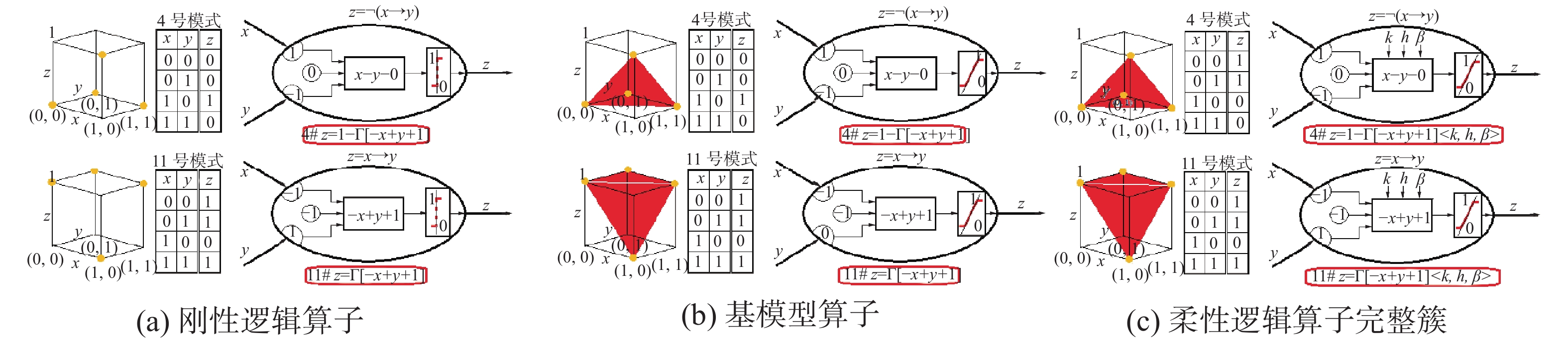Download: 图 17 非蕴涵1模式和蕴涵1模式 Fig. 17 N-IMP-1 pattern and IMP-1 pattern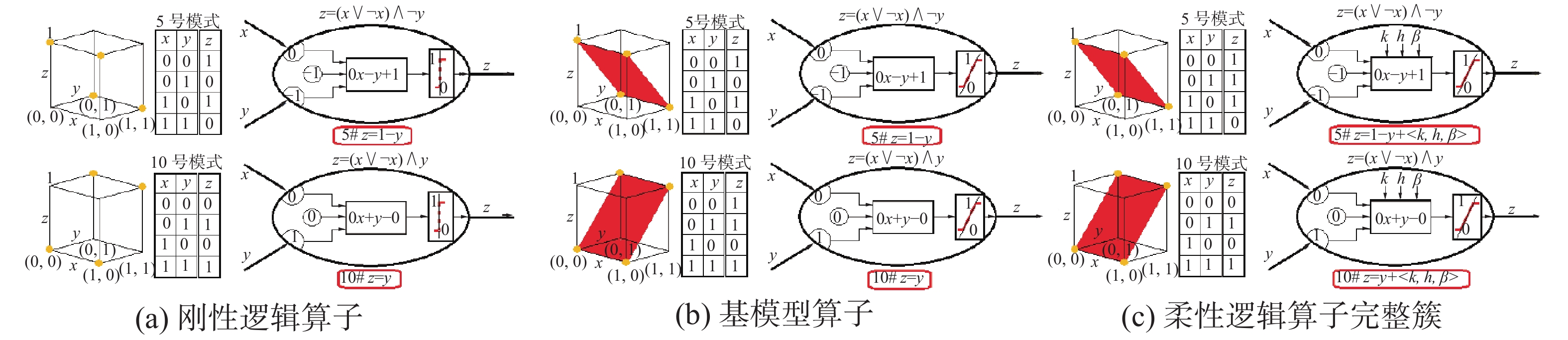Download: 图 18 非y模式和指y模式 Fig. 18 NOT-y pattern and IND-y pattern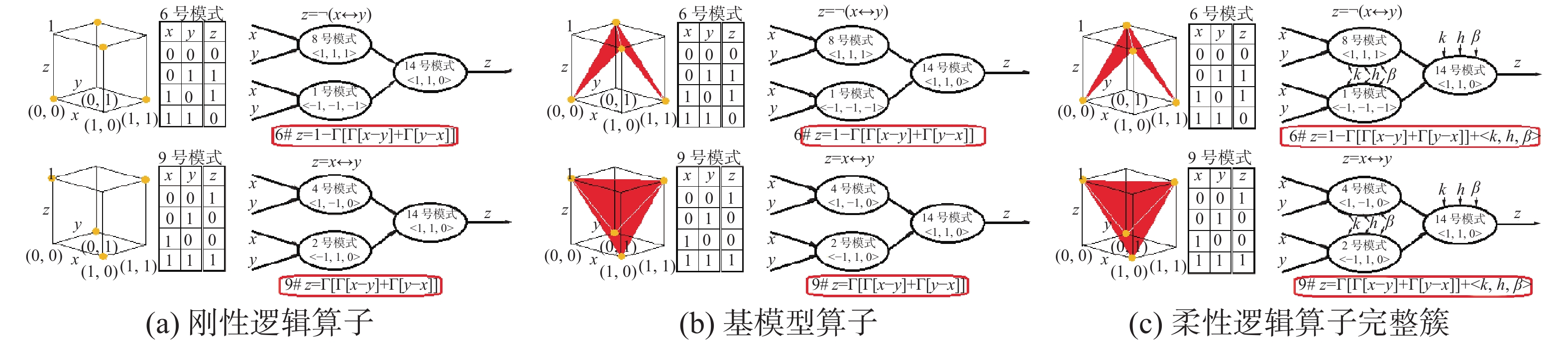Download: 图 19 非等价模式和等价模式 Fig. 19 N-EQU pattern and EQU pattern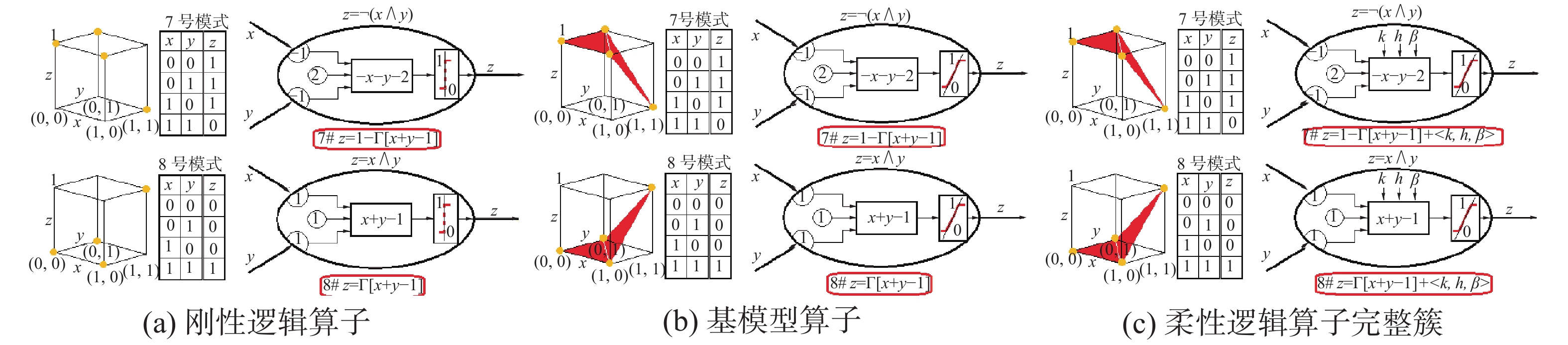Download: 图 20 非与模式和与模式 Fig. 20 N-AND pattern and AND pattern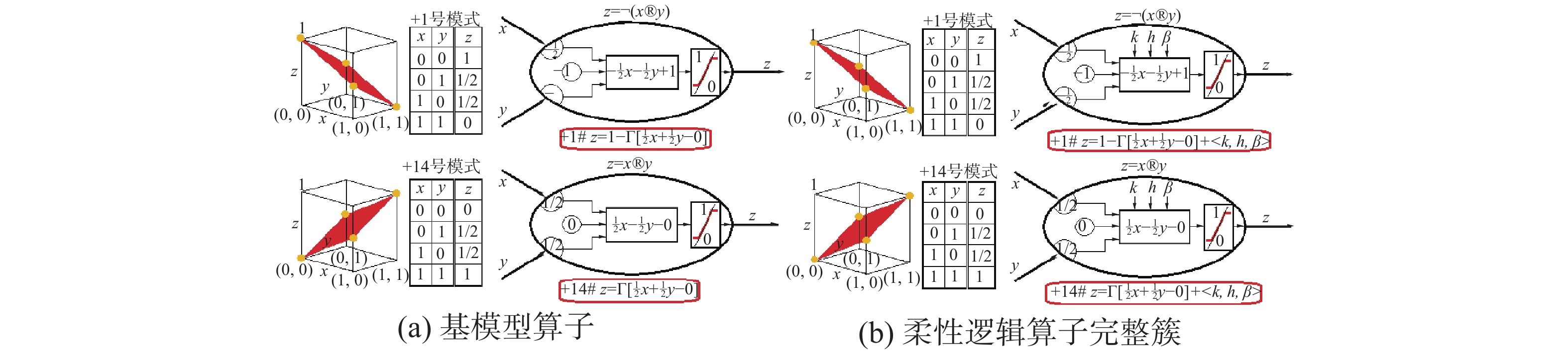Download: 图 21 非平均模式和平均模式 Fig. 21 N-AVE pattern and AVE pattern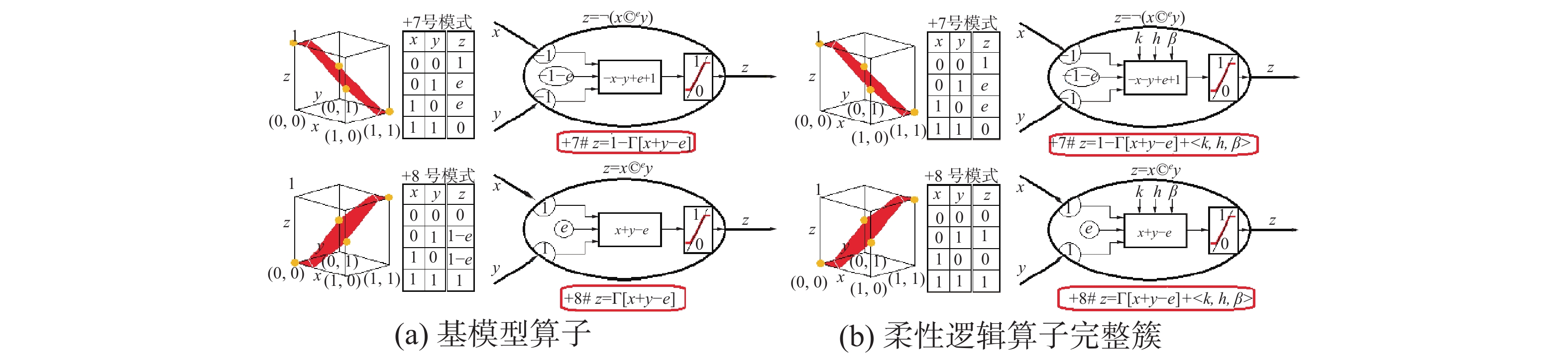Download: 图 22 非组合模式和组合模式 Fig. 22 N-COM pattern and COM pattern

 $\begin{split} S(x,y,k,h,\beta ) = & \Bigl( {1 - \left( {\max \left( {0,2\beta {{\left( {1 - {x^n}} \right)}^m} + } \right.} \right.} \\ & {\left. {{{\left. {\left. {2(1 - \beta ){{\left( {1 - {y^n}} \right)}^m} - 1} \right)} \right)}^{1/m}}} \right)^{1/n}} \end{split}$

β=0.5时权系数的影响消失，S(x, y, k, h)=(1−(max(0, (1−xn)m+(1−yn)m−1))1/m)1/n

k=0.5时误差系数的影响消失，S(x, y, h)=(1−(max(0, (1−x)m+(1−y)m−1))1/m

S(x, y, h)有4个特殊算子: Zadeh或算子S(x, y, 1)=max(x, y) ；概率或算子S(x, y, 0.75)=x+yxy；有界或算子S(x, y, 0.5)=min(1, x+y)；突变或算子S(x, y, 0)=ite{max(x, y)|min(x, y)＝0;1}。

 $N(x, k)=\left(1-x^{n}\right)^{1 / n}$

 $I(x,y,k,h,\beta ) = {(\min (1,\;1 - 2\beta {x^{nm}} + 2(1 - \beta ){y^{nm}}))^{1/mn}}$

β＝0.5时权系数的影响消失，I(x, y, k, h)=(min(1, 1−xnm+ynm))1/mn

k＝0.5时误差系数的影响消失，I(x, y, h)=(min(1, 1−xm+ym))1/m

I(x, y, h)有4个特殊算子: Zadeh蕴涵I(x, y, 1)=ite{1|xy; y}；概率蕴涵I(x, y, 0.75)=min(1, y/x) ；有界蕴涵I(x, y, 0.5)=min(1, 1−x+y)；突变蕴涵I(x, y, 0)=ite{y|x=1; 1}。

 $\begin{split} Q(x, y, k, h, \beta )= & \text { ite }\left\{\left(1+\left|2 \beta x^{n m}-2(1-\beta) y^{n m}\right|\right)^{1 / m n} | m \leqslant 0\right.;\\ & \left(1-\left|2 \beta x^{n m}-2(1-\beta) y^{n m}\right|\right)^{1 / m n} \} \end{split}$

β=0.5时权系数的影响消失，Q(x, y, k, h)=ite{(1+|xnmynm|)1/mn|m≤0; (1−|xnmynm|)1/mn}；

k=0.5时误差系数的影响消失，Q(x, y, h)=ite{(1+|xmym|)1/m|m≤0; (1−|xmym|)1/m}。

Q(x, y, h)有4个特殊算子: Zadeh等价Q(x, y, 1)=ite{1|x=y;min(x, y)}；概率等价Q(x, y, 0.75)= min(x/y, y/x)；有界等价Q(x, y, 0.5)=1−|xy|；突变等价Q(x, y, 0)=ite{x|y=1;y|x=1;1}。

 $T\left( {x,y,k,h,\beta } \right) = {\left( {\max \left( {0,2\beta {x^{nm}} + 2(1 - \beta ){y^{nm}} - 1} \right)} \right)^{1/mn}}$

β=0.5时权系数的影响消失，T(x, y, k, h)=(max(0, xnm+ynm−1))1/mn

k=0.5时误差系数的影响消失，T(x, y, h)= (max(0, xm+ym−1))1/m

T(x, y, h)有4个特殊算子: Zadeh与算子T(x, y, 1) =min(x, y)；概率与算子T(x, y, 0.75)=xy；有界与算子T(x, y, 0.5)=max(0, x+y−1)；突变与算子T(x, y, 0)=ite{min(x, y)|max(x, y)=1; 0}。

 $M(x,y,k,h,\beta ) = {(1 - {(\beta (1 - {x^n}){^m} + (1 - \beta)(1 - {y^n}){^m})^{1/m}})^{1/n}}$

β＝0.5时权系数的影响消失，M(x, y, k, h) =(1−((1−xn)m+(1−yn)m)1/m)1/n

k＝0.5时误差系数的影响消失，M(x, y, ···, h)=1−((1−x)m+(1−y)m)1/m

M(x, y, h)有4个特殊算子: Zadeh平均M(x, y, 1) =max(x, y)；概率平均M(x, y, 0.75)= 1−((1−x)(1−y))1/2 ；有界平均M(x, y, 0.5)=(x+y)/2；突变平均M(x, y, 0)=min(x, y)。

 \begin{aligned} & {C^e}(x,y,k,h,\beta ) = {\rm{ite}}\{ \min (e,\;(\max (0,\;2\beta {x^{nm}} + \\ & 2(1 - \beta ){y^{nm}} - {e^{nm}}){)^{1/mn}}|2\beta x + 2(1 - \beta )y2e;\\ & (1 - (\min (1 - {e^n},\;(\max (0,\;2\beta {(1 - {x^n})^m} + \\ & 2(1 - \beta ){(1 - {y^n})^m} - {(1 - {e^n})^m}){)^{1/m}}){)^{1/n}})|\\ & 2\beta x + 2(1 - \beta )y > 2e;e\} ; \end{aligned}

β＝0.5时权系数的影响消失，Ce(x, y, k, h)= ite{min(e, (max(0, xnm+ynmenm))1/mn|x+y<2e; (1−(min (1−en, (max(0, (1−xn)m+(1−yn)m−(1−en)m))1/m))1/n))|x+y>2e;e};

k＝0.5时误差系数的影响消失，Ce(x, y, h)=ite{min(e, (max(0, xm+ymem))1/m|x+y<2e; (1−(min(1−e, (max(0, (1−x)m+(1−y)m−(1−e)m))1/m)|x+y>2e; e}。

Ce(x, y, h)有4个特殊算子: Zadeh组合Ce(x, y, 1)=ite{min(x, y)|x+y<2e; max(x, y)|x+y>2e;e}；概率组合Ce(x, y, 0.75)=ite{xy/e|x+y<2e;(x+yxye)/(1−e)|x+y>2e;e}；有界组合Ce(x, y, 0.5)=Γ[x+ye]；突变组合Ce(x, y, 0)=ite{0|x, y<e; 1|x, y>e; e}。Download: 图 23 在组合运算中决策阈值e的影响 Fig. 23 Influence of decision threshold e on the combinatorial operation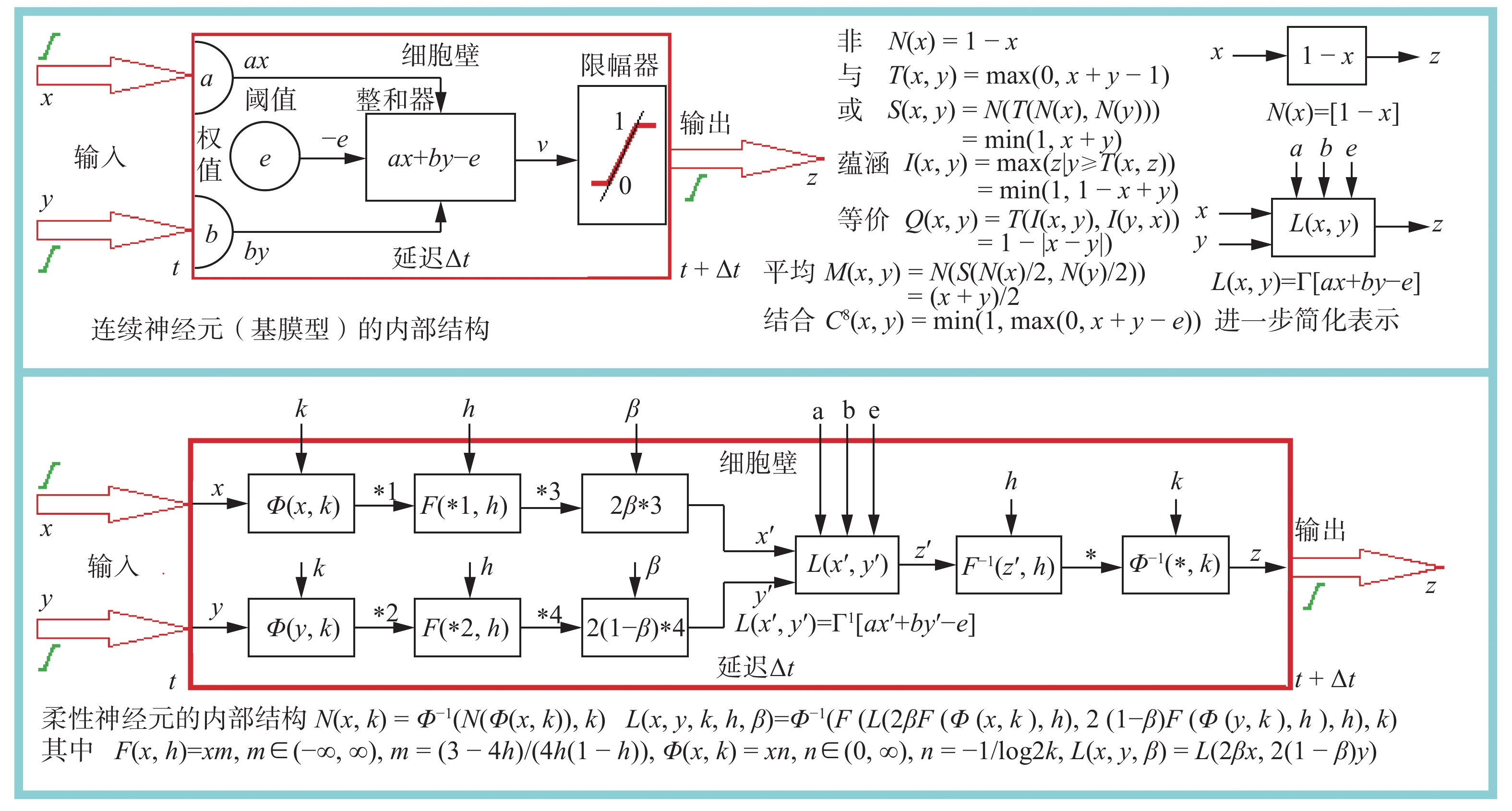Download: 图 24 柔性神经元的信息处理全过程 Fig. 24 Entire process of information processing of flexible neurons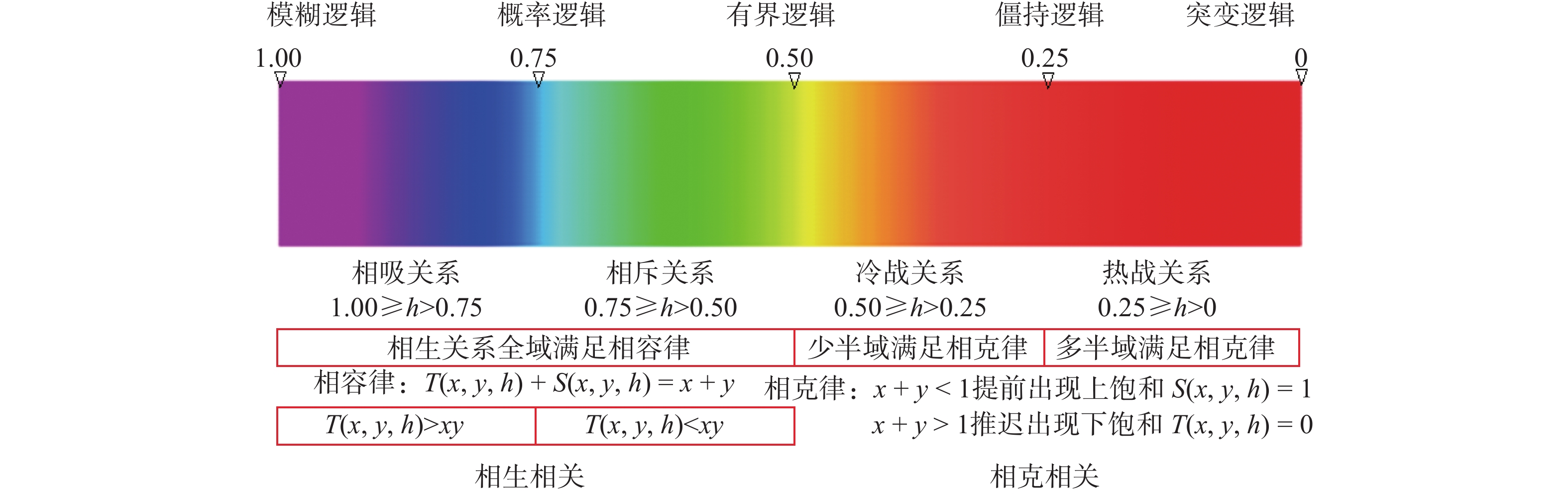Download: 图 25 一维命题逻辑谱L(x, y, h)的包容性 Fig. 25 Inclusiveness of the one-dimensional propositional logic spectrum L(x, y, h)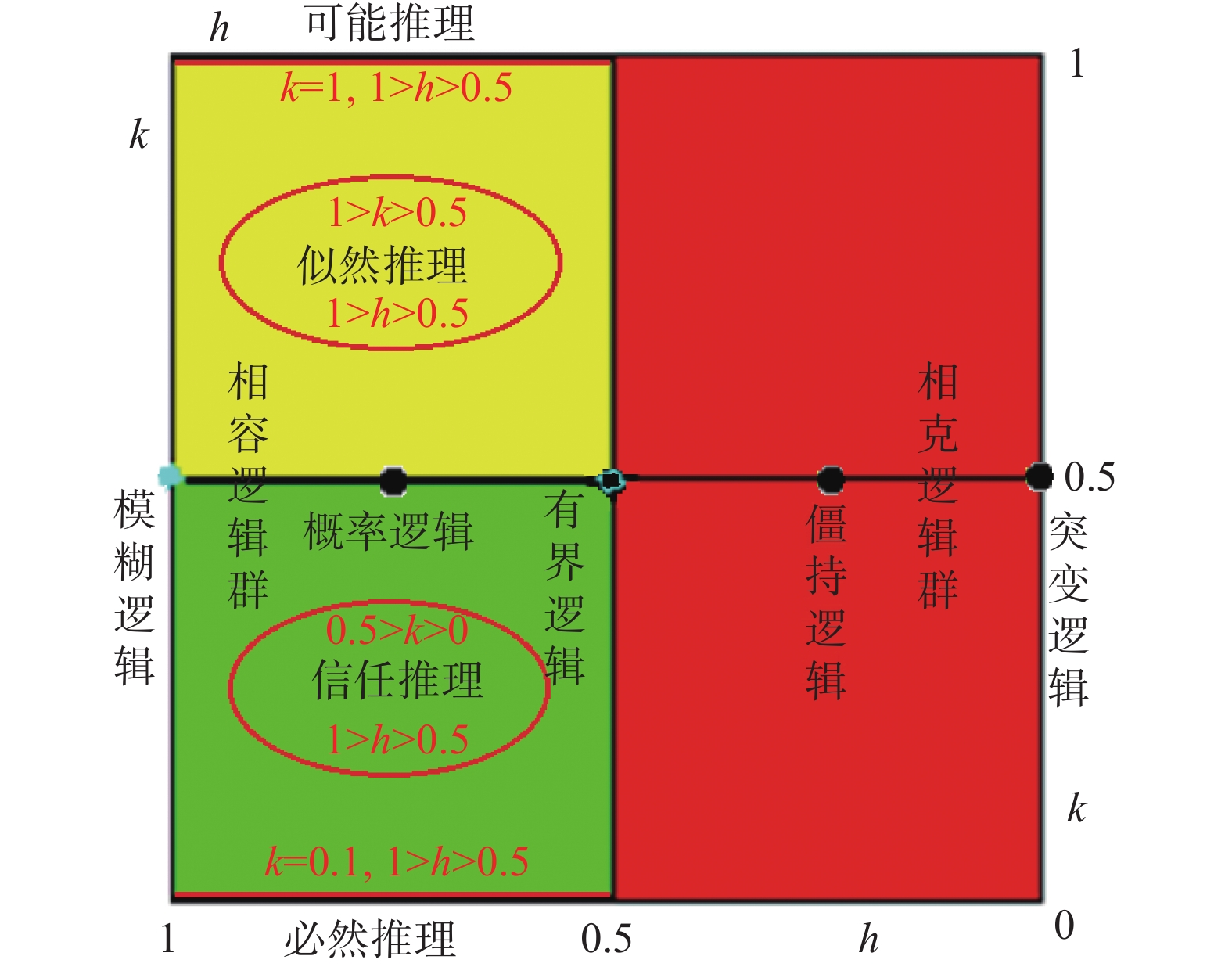Download: 图 26 二维命题逻辑谱L(x, y, k, h)的包容性 Fig. 26 Inclusiveness of the two-dimensional propositional logic spectrum L(x, y, k, h)
2.4 柔性命题逻辑算子簇的完备性和应用针对性 2.4.1 柔性命题逻辑算子簇的完备性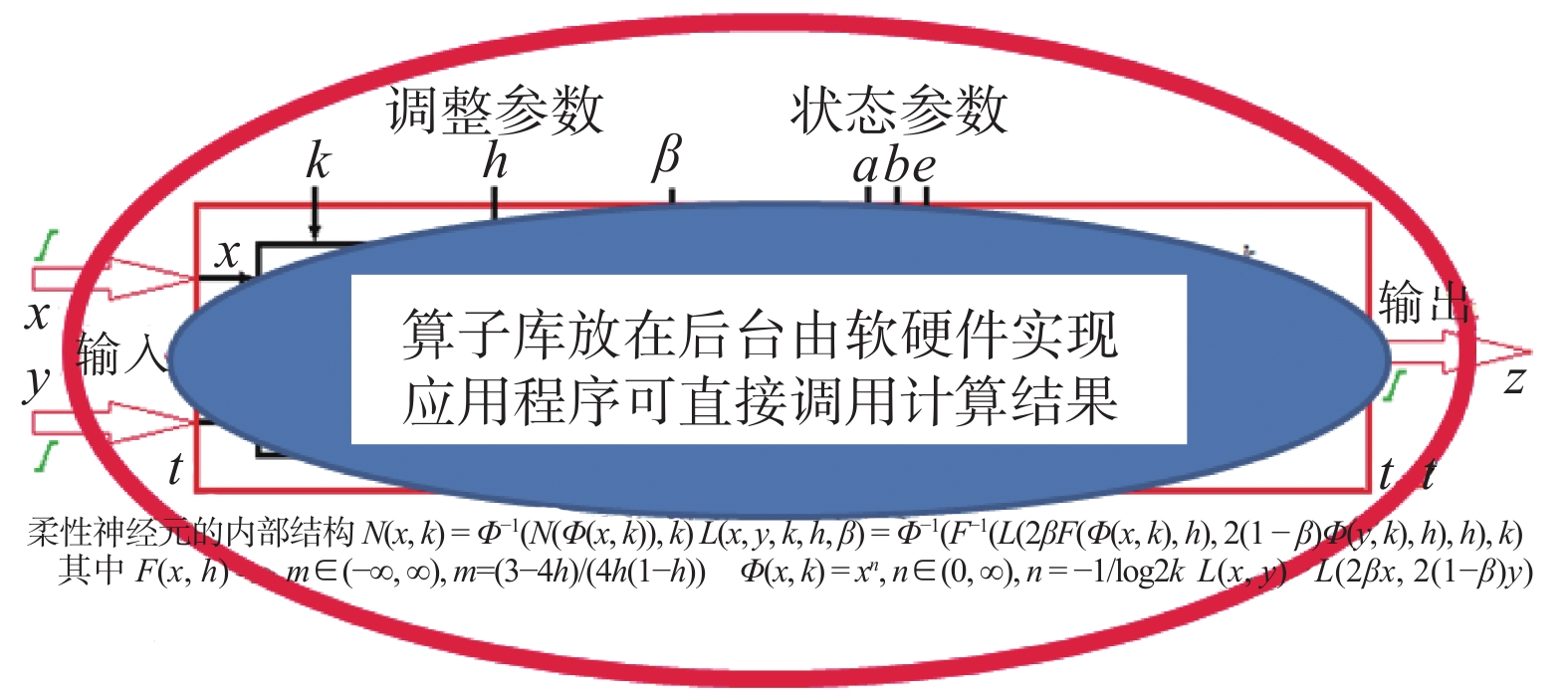Download: 图 27 使用柔性逻辑算子如同查三角函数表一样方便 Fig. 27 Using flexible logic operators is as convenient as looking at trigonometric functions

1)二元布尔信息处理只有16种信息处理模式，二元柔性基模型信息处理只有20种信息处理模式，三元以上的信息处理可以用多个二元信息处理来表示，所以这20种模式是完备的，它们由信息处理模式的状态参数<a, b, e>唯一确定。

2)在基模型中已经包含了命题真度的不确定性x∈[0, 1]，它可以从最大值1连续变化到最小值0，中间没有遗漏，具有完备性。

3)能够对各种基模型产生调控作用的不确定性参数只有3个，它们是k∈[0, 1]，h∈[0, 1]，β∈[0, 1]，另外能对组合运算基模型产生调控作用的不确定性还有一个e∈[0, 1]，它们形成的算子完整簇都能够从最大可能的算子连续变化到最小可能的算子，中间没有遗漏，具有完备性，它们可由模式内的不确定性调整参数<k, h, β>唯一确定，(另一个不确定性调整参数e是两边共用的参数)。

2.4.2 柔性命题逻辑算子的应用针对性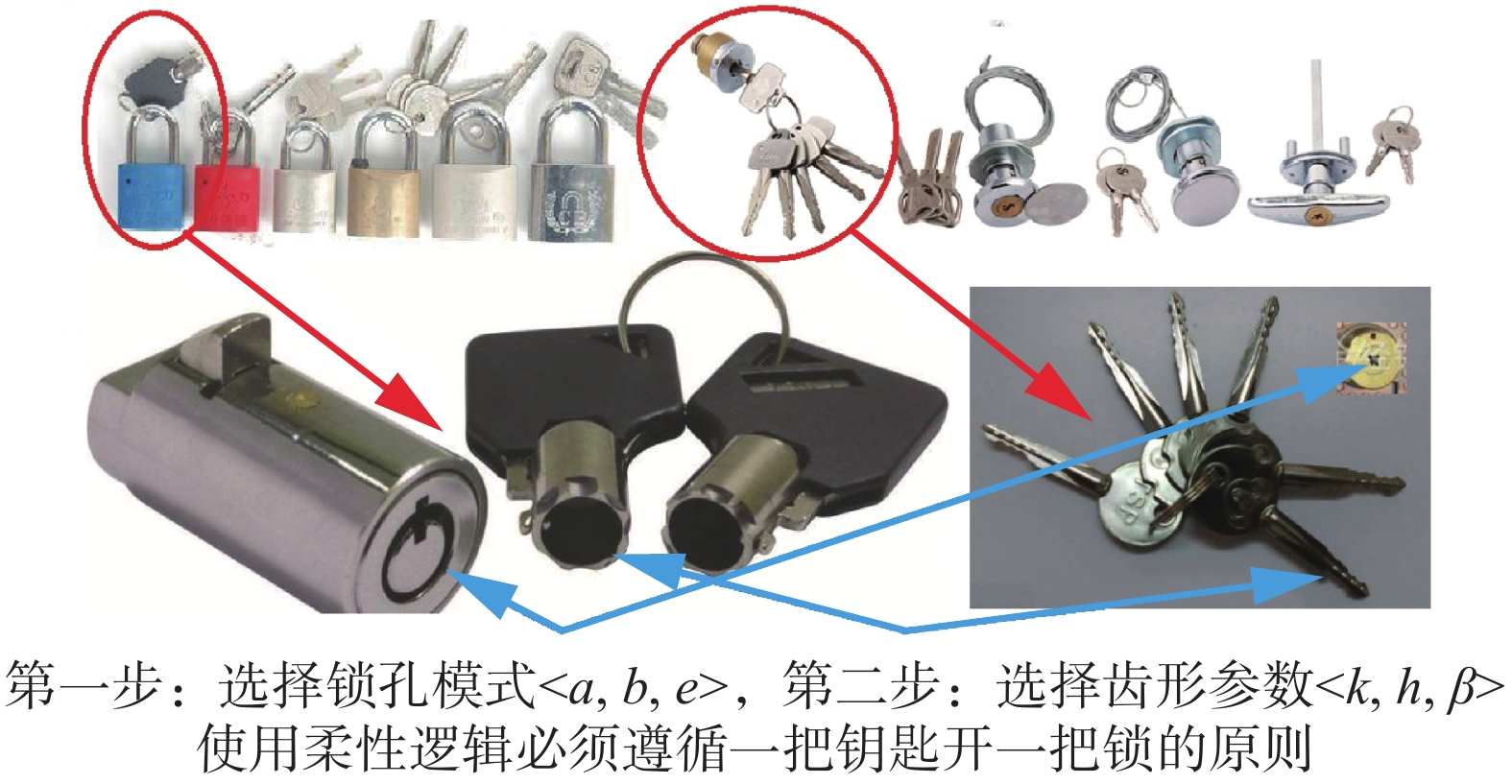Download: 图 28 在柔性逻辑中需要一把钥匙开一把锁 Fig. 28 A key needed to open a lock in the flexible logic

3 确保智能信息处理过程和结果的可解释性

3.1 智能信息处理必须正视各种不确定性和演化过程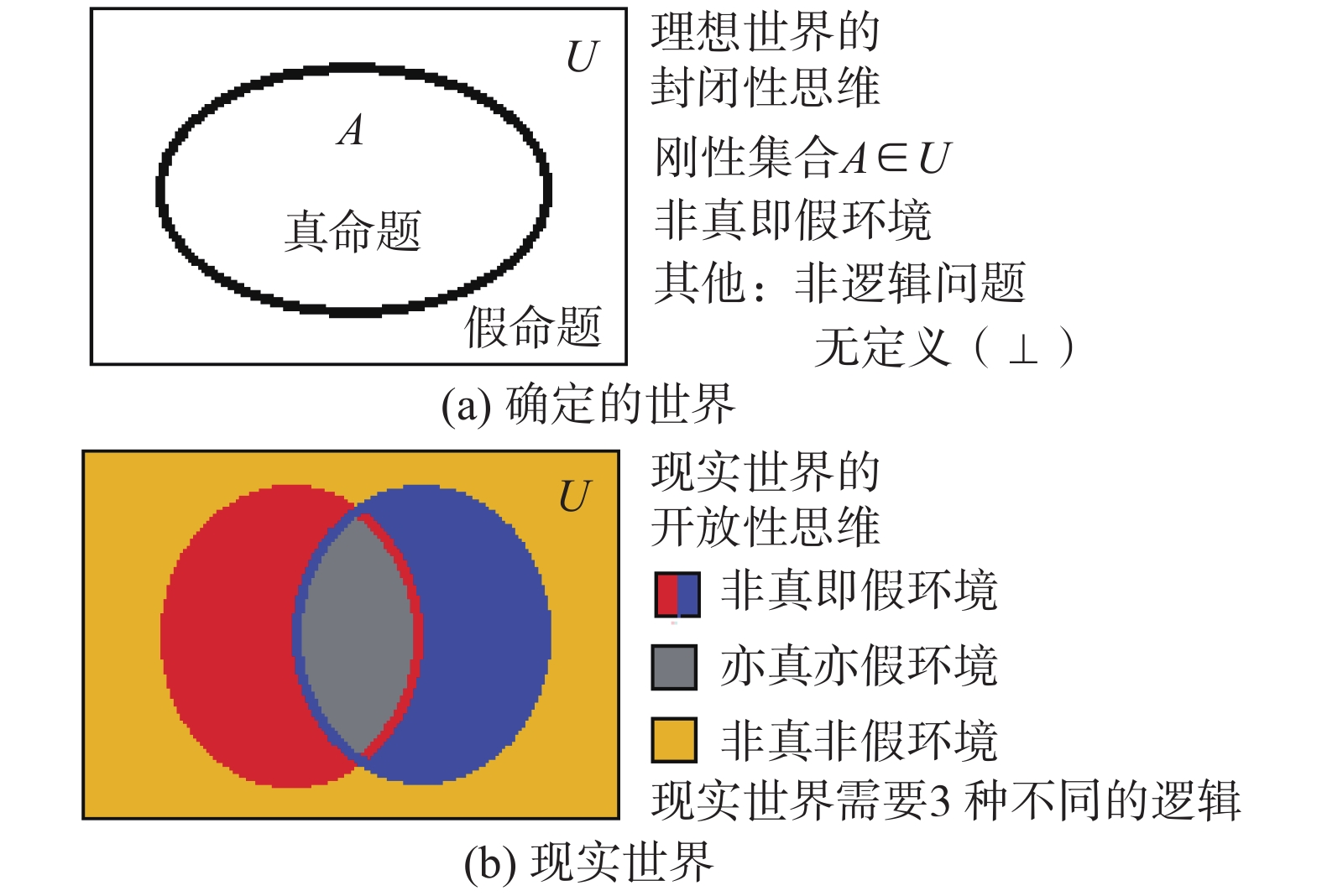Download: 图 29 不同世界有不同的逻辑环境 Fig. 29 Different worlds have different logical environmentsDownload: 图 30 智能信息处理需要的完整逻辑谱 Fig. 30 Complete logic spectrum required for intelligent information processingDownload: 图 31 多层次多粒度的知识结构图 Fig. 31 Multilevel and multigranularity knowledge structure diagrams

3.2 如何精确确定各种柔性逻辑算子的有关参数

 $f\left( {{x_1},{x_2},{x_3}} \right) = {f_j}\left( {{f_i}\left( {{x_1},{x_2}} \right),{x_3}} \right),i,j \in \{ 0,1,2,3, \cdots ,15\} \text{。}$

1)当i=j

z=fi(fi(x1, x2), x3)=fi(x1, x2, x3), i∈{0, 1, 2, 3, ···, 15}

 \begin{aligned} {\textit{z}} = & f\left( {{x_1},{x_2},{x_3},{x_4}} \right) = {f_k}\left( {{f_i}\left( {{x_1},{x_2}} \right),{f_j}\left( {{x_3},{x_4}} \right)} \right), \\ & i,j,k \in \{ 0,1,2,3, \cdots ,15\} \end{aligned}

2)当i=j=k

z=fi(fi(x1, x2), fi(x3, x4))=fi(x1, x2, x3, x4), i∈{0, 1, 2, 3, ···, 15}

 $z = \Gamma [{{a}}x + {{b}}y - {{e}}],\quad x,y,z \in [0,1]$

1)误差系数k的确定。在柔性非运算N(x, k)中，k是不动点N(k, k)=k，所以在K1中非模式的因果关系数据中，如果发现有输入和输出相等的情况x=z=k，这个k就是误差系数，k=0.5表示没有误差。如果没有发现完全相等的数据，可以寻找尽可能接近的数据对<x; z>，这时的k≈(x+z)/2。

2)广义相关系数h的确定。根据K1中柔性与运算T(x, y, h)的因果关系数据，确定广义相关系数h的方法主要有两种：与算子体积法和x=y主平面上的标准尺测量法。

①与算子体积法：

 $\begin{array}{c} h = 3\int_0^1 {\int_0^1 T } (x,y,h){\rm{d}}x{\rm{d}}y \approx 3{\Sigma _i}{\Sigma _j}T\left( {{x_i},{y_j},h} \right)/mn,\\ i = 1,2,3, \cdots ,m,j = 1,2,3, \cdots ,n \end{array}$

x=y主平面上的标准尺测量法：

x=y平面上绘制z=T(x, x, h)曲线，这个曲线与x=0.5的垂直线或者z=0的水平线的交点位置(相对于图32中垂直分布的h标准尺来说)，就是这个与算子的广义相关系数h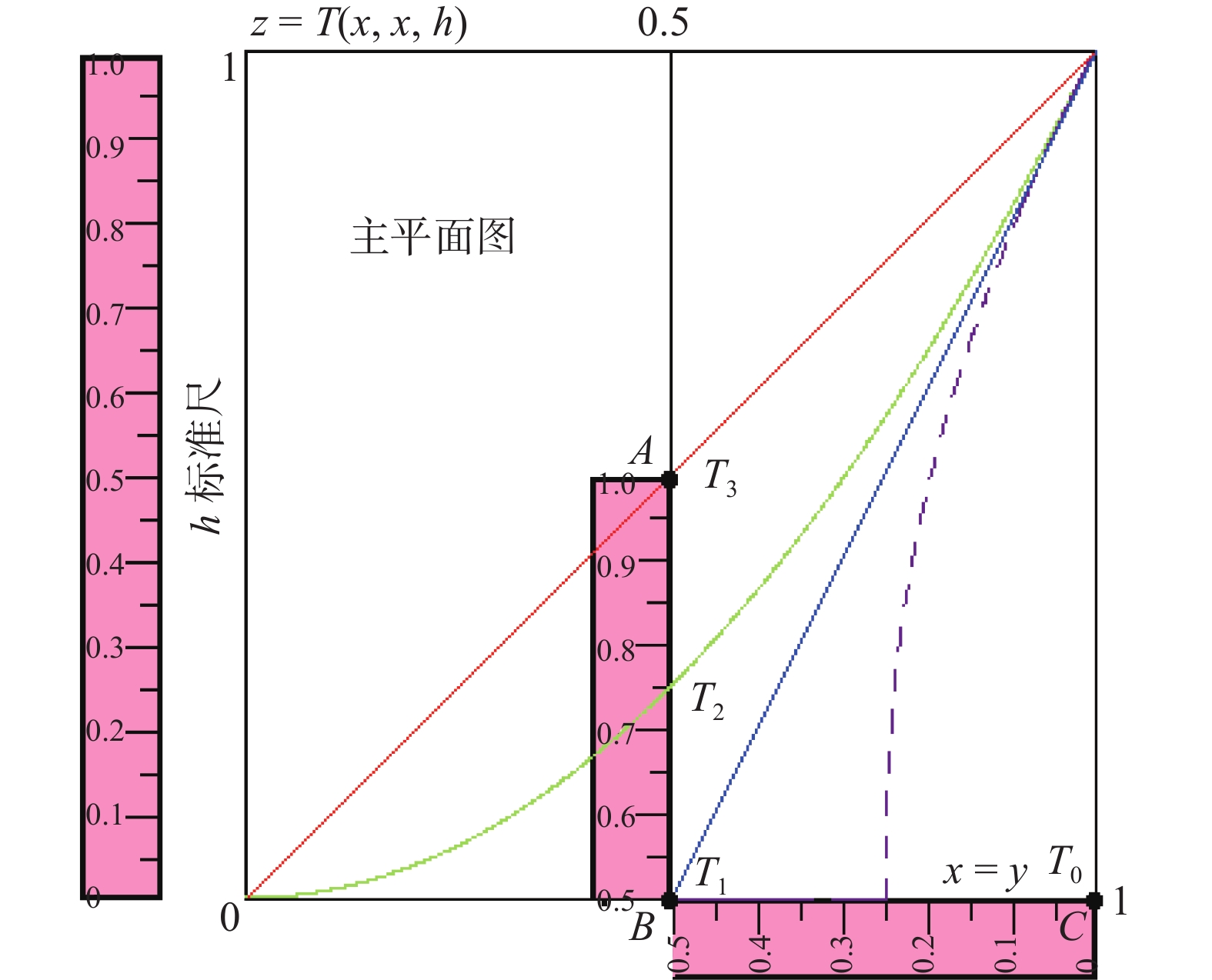Download: 图 32 h的标准尺测量法 Fig. 32 The standard measurement of h

3)相对权重系数β的确定。根据K1中柔性平均运算M(x, y, h, β)的因果关系数据，确定相对权重系数β的方法在M(x, y, 0.5, β)时比较方便，因为这时的M(1, 0, 0.5, β)=β(见图33)，而在K1中寻找这样的特殊数据是不困难的。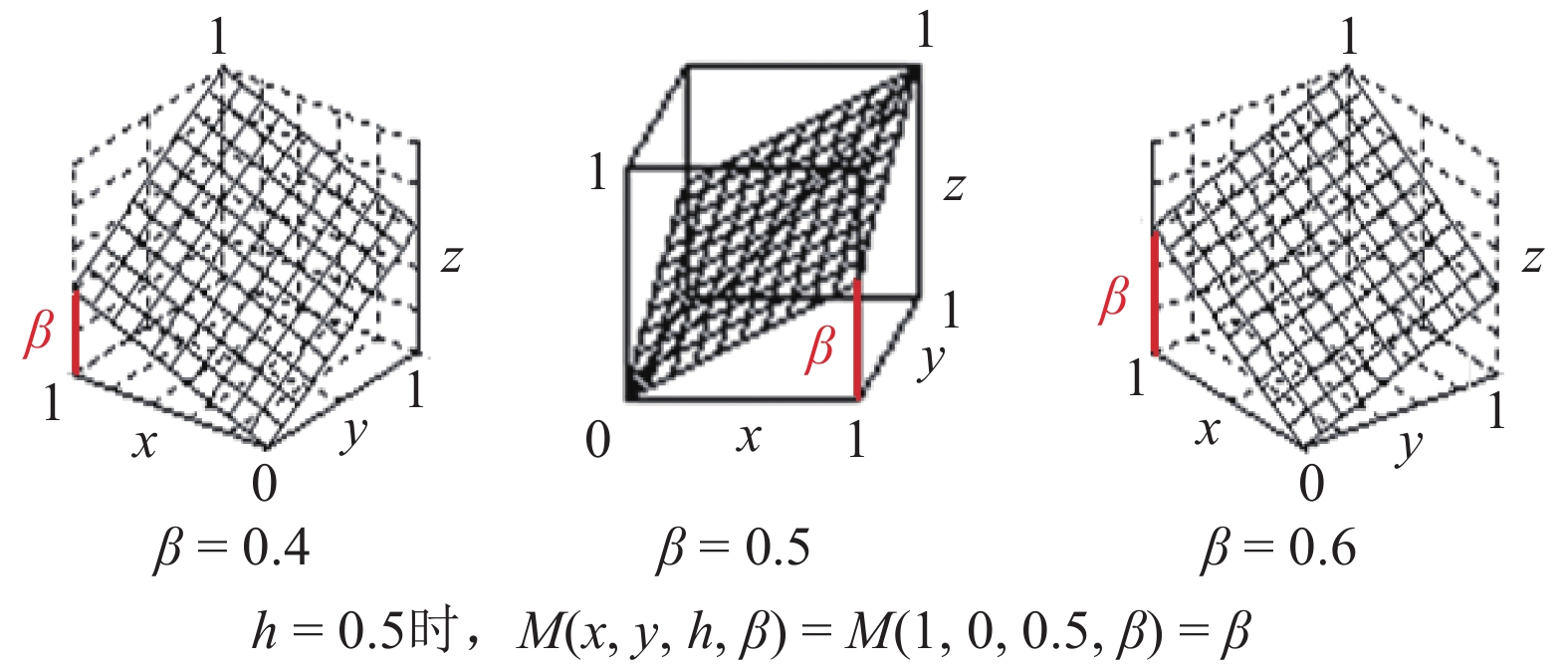Download: 图 33 相对权重系数β的确定 Fig. 33 Determination of coefficient β of the relative weight

4)组合运算中决策阈值系数e的确定。根据K1中柔性组合运算Ce(x, y, h)的因果关系数据，确定决策阈值系数e的方法在Ce(x, y, 0.5)中比较方便，因为这时组合运算的下平台区最大边界线L正好是满足x+y=e的一条直线(见图34)，这样的数据在K1中很容易找到。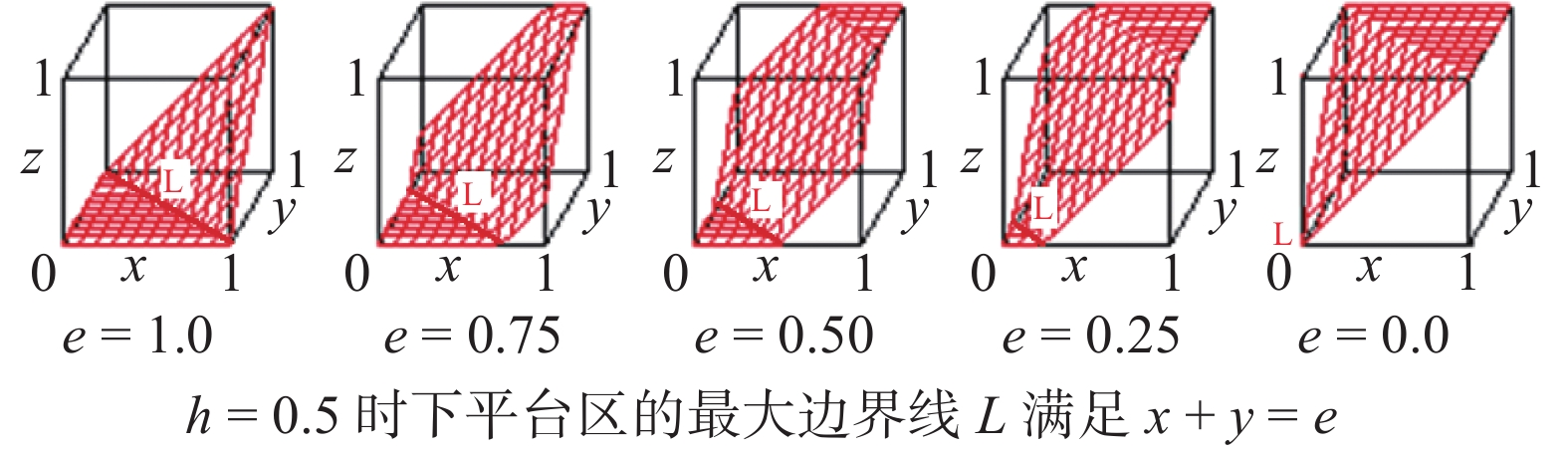Download: 图 34 组合运算中决策阈值系数e的确定 Fig. 34 Determination of decision threshold coefficient e in the combinatorial operation

4 结束语

  何华灿. 人工智能导论[M]. 西安: 西北工业大学出版社, 1988. 11-15 (0)  何华灿. 泛逻辑学理论: 机制主义人工智能理论的逻辑基础[J]. 智能系统学报, 2018, 13(1): 19-36. HE Huacan. Universal logic theory: logical foundation of mechanism-based artificial intelligence theory[J]. CAAI transactions on intelligent systems, 2018, 13(1): 19-36. (0)  何华灿, 何智涛. 从逻辑学的观点看人工智能学科的发展[M]//. 涂序彦. 人工智能: 回顾与展望. 北京: 科学出版社, 2006: 77-111. (0)  谭铁牛. 人工智能: 天使还是魔鬼?[EB/OL]. (2018-06-13)[2019-04-10]. http://www.sohu.com/a/235446077_453160. (0)  何华灿, 王华, 刘永怀, 等. 泛逻辑学原理[M]. 北京: 科学出版社, 2001. (0)  HE Huacan, WANG　Hua, LIU Yonghuai, et al. Principle of universal logics[M]. Beijing: Science Press, 2006. (0)  HE Huacan. The outline on continuous-valued logic algebra[J]. International journal of advanced intelligence, 2012, 4(1): 1-30. (0)  张文修. 不确定性推理原理[M]. 西安: 西安交通大学出版社, 1996. (0)  伊利亚·普里高津. 确定性的终结: 时间、混沌与新自然法则[M]. 湛敏, 译. 上海: 上海科技教育出版社, 1998: 1–12 (0)  张金成. 逻辑及数学演算中的不动项与不可判定命题(I)[J]. 智能系统学报, 2014, 9(4): 499-510. ZHANG Jincheng. Fixed terms and undecidable propositions in logical and mathematical calculus (I)[J]. CAAI transactions on intelligent systems, 2014, 9(4): 499-510. (0)  何华灿. S型超协调逻辑中的一项重大研究突破: 评张金成《逻辑及数学演算中的不动项与不可判定命题》[J]. 智能系统学报, 2014, 9(4): 511-514. DOI:10.3969/j.issn.1673-4785.2014.04.022 (0)  张金成. 悖论、逻辑与非Cantor集合论[M]. 哈尔滨工业大学出版社, 2018. (0)  钟义信. 机制主义人工智能理论: 一种通用的人工智能理论[J]. 智能系统学报, 2018, 13(1): 2-18. ZHONG Yixin. Mechanism-based artificial intelligence theory[J]. CAAI transactions on intelligent systems, 2018, 13(1): 2-18. (0)  汪培庄. 因素空间理论: 机制主义人工智能理论的数学基础[J]. 智能系统学报, 2018, 13(1): 37-54. WANG Peizhuang. Factor space-mathematical basis of mechanism based artificial intelligence theory[J]. CAAI transactions on intelligent systems, 2018, 13(1): 37-54. (0)